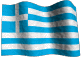Short-term Earthquake Prediction Based on Seismic Precursory Electric  Signals Recorded on Ground Surface.

 CONTENTS 4.3.1. 4.3.2. 4.3.3. 4.3.4. 4.3.5. 4.3.5.a. 4.3.5.b. 4.3.5.c. 4.3.6. 4.3.6.1. 4.3.6.2. 4.3.6.3. 4.3.6.4. 4.3.7. 4.3.7.1. 4.3.7.2.4.3. Magnitude determination.

4.3.1. Introduction.

There is a limited number of studies, which refer to the magnitude, in the Earthquake Prediction literature. The past seismic history (statistically treated) plus the dimensions of the studied, tectonic features of a seismogenic area provided some vague clues for the probable magnitude of the imminent strong EQ.
A physical model that will account for the determination of the prediction parameters of a strong EQ, is missing, in a way, from the seismological community.
The starting point of this topic is that different earthquake prediction parameters, most probably, require a different physical model which will be applied for its determination. Moreover, these physical models should not contradict but comply with each other. For the case of magnitude determination the “Lithospheric Seismic Energy Flow Model”, which is introduced in section (2.5.1), is used. This model is based on the balance of the absorbed and released energy, in the lirhospheric seismogenic area, where an earthquake will occur in the future.
The rate of seismic energy release, as a function of an inverse power of the time, remaining to the main, seismic event, has been proposed and applied to back-analyses of several foreshock main sequences by Varnes (1987a, b).
In these papers, Varnes analyses the accelerating, precursory seismic activity in terms of seismic moment release. A simple, in time (t), empirical power law failure function was postulated (Bufe and Varnes, 1993) that related the parameters of the remaining time (tc-t) to the occurrence of the imminent, strong EQ and its corresponding magnitude (M) to the seismic moment release (the term accumulated Benioff strain is often used). Main (1995) studied the earthquakes from the point of view of a critical phenomenon.
In the same area of Statistical Physics (Main, 1996), Bowman et al. (1998) showed that the cumulative, seismic strain release increases, as a power law time to failure, before the final event. Papazachos et al. (2000) used the same methodology to study the Benioff strain rate release of the Aegean area. Moreover, an estimate for the timing of an imminent strong EQ was achieved with an accuracy of +/- 1.5 years, by using the same methodology for EQs of the Aegean area (Papazachos et al. 2001). The time to failure model was used, as a technique, in which a failure function is fitted to a time series of accumulated Benioff strain, by Di Giovambattista and Tyupkin (2001), in order to analyze the relation of the time-to-failure model to the hypothesis of fractal structure of seismicity. This method was applied on laboratory rock samples fracturing and real strong EQs of Kamchatka and Italy, as well.
In the early papers, that deal with the “Benioff strain - accelerated deformation – critical point” method, the studied seismogenic area was related to a circle of an optimum radius R and, lately, to an elliptical area (Papazachos et al. 2001).
The common feature, of all these papers, is the absence of a physical model that accounts for the well-known time to failure equation which is used very often. This equation is an empirical one and derives from the Statistical Physics, applied to other fields of applied science. Moreover, no tectonic information is taken into account, as far as it concerns the under study seismogenic area.
In this section, two main, new features are presented, for the understanding of the methodology. The first one is a postulated physical model that justifies the accelerated energy release method, while the second one is the deep lithospheric fracture zones (Thanassoulas, 1998) where strong EQs are generated and constrain the extent of the seismogenic area to be studied.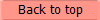4.3.2. Theoretical model analysis.

It is well known that the stress, built up, in a focal area, is a very slow process which follows closely the motion of the lithospheric plates. It takes a long period of time (many years) to reach the point when an earthquake will occur because of the rock fracturing.
In normal conditions, the stored energy is discharged through the normal (background) small magnitude seismicity of the area. When a strong earthquake is in preparation, this normal seismicity is decreased, for a certain time period, and therefore, the detected seismic “quiescence” was used as a precursory indicator.
The latter is presented in the following figure (4.3.2.1). The cumulative number of seismic events, plotted, against time, deviates from the form of a linear function and resumes its normal one after the occurrence of the expected EQ.Fig. 4.3.2.1. Example of a seismic quiescence precursor.

Although this methodology gave successful results, with the way it treated the seismological data, it does not answer the question of the magnitude, neither the time of occurrence and it still remains in the domain of statistical methods. On the other hand, in terms of Physics, the same phenomenon can be studied from the energy transfer and balance point of view of the regional seismogenic area.
An open, physical system, that constantly absorbs energy, remains in a dynamic equilibrium when the in-flow energy is equal to out-flow energy, otherwise that physical system, should “explode”, at a certain moment, releasing the excessive, stored energy, in a short time.
In the case of seismicity, the open physical system, in question, is the regional area of the lithosphere where the EQ will occur. Practically, the lithosphere is at a state of critical dynamic equilibrium, or it is teetering on the edge of instability, with no critical length scale (Bak, Tang and Wiesenfeld 1988; Bak 1996) as far as it concerns in-flow and out-flow of strain energy. The following figure (4.3.2.2) shows the postulated “energy - flow model” in such a condition.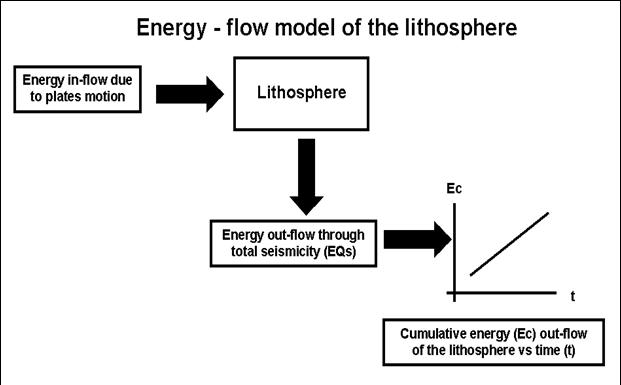Fig. 4.3.2.2. Energy – flow model of the lithosphere and cumulative energy out-flow (Ec), as a function of time.

Theoretically, following this working physical model, the cumulative energy release in a seismogenic area, during a normal, seismic period, should be a linear function (equation 2.5.1.8) of time, due to energy conservation law of Physics. This is demonstrated in the lower right part of the figure (4.3.2.2).
The cumulative energy (Ec) release function vs. time (t), for a specific, seismogenic area, can be determined by converting the magnitude of each, recorded, EQ in the past, into energy, using any suitable formula that exists in the seismological literature. In the present work was used the following empirical formula (Tselentis, 1997):

LogE = 11.8 + 1.5Ms                                                                 (4.3.2.1)
Where:

E is the energy, calculated, in ergs.

Ms is the magnitude of each EQ, in Richter scale.

By applying this procedure to all EQs that occurred during the period of study, the cumulative energy Ec can be calculated and therefore, the graph Ec = f(t) can be constructed.
As an example for the results, expected, over a seismogenic but of normal energy release period, the following figure (4.3.2.3) is presented. In a 20 years period of time that preceded the 1997/11/18, Ms 6.6R EQ in Greece, two distinct, normal energy release periods can be identified. The first one spans from 1 to 43, while the second spans from 67 to 205, in time scale (months). In these two periods the cumulative energy out-flow, due to normal seismicity, is that of the form of a linear function in time.Fig. 4.3.2.3. Cumulative energy out-flow graph vs. time, for the seismogenic area of the EQ, on 18/11/1997 (Ms=6.6R) in Greece. Time period: 1977 – 1997.

Although this is the normal condition of the lithosphere, due to certain, mostly frictional, mechanical reasons, the seismogenic area, at a specific time, “locks”. At this state the normal seismicity decreases and therefore the energy out-flow decreases. As an immediate result, the in-flow energy is stored in the seismogenic area. This stored excessive energy will be released in the future as an EQ of corresponding magnitude, at the time, when the seismogenic area “unlocks” during rock rupture. This is demonstrated in the following figure (4.3.2.4).Fig. 4.3.2.4. Theoretical cumulative energy out-flow “lock” model.

In figure (4.3.2.4), the two black arrows indicate the start and the end of the “lock period”. At the end of the “lock period”, the imminent EQ takes place. Its magnitude is calculated by the two energy levels E1, 2 using formula (4.3.2.1). After the expected EQ has occurred, the seismogenic region resumes its normal energy release rate, based on seismicity of small EQs.
A more general case is the one, in which the seismogenic area is always in critical strain conditions (mechanically locked) and earthquakes occur randomly in time, due to mainly frictional, mechanical reasons. This is demonstrated in the following figure (4.3.2.4a).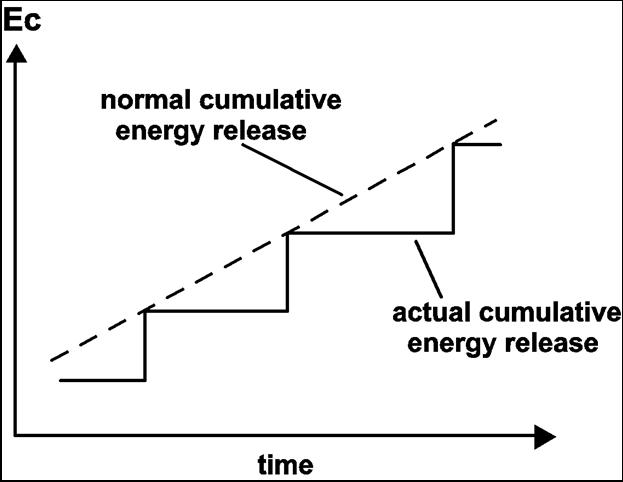Fig. 4.3.2.4a. Step-wise cumulative, seismic energy release sketch presentation. The dashed line indicates the corresponding, normal, cumulative energy out-flow under stable conditions. Step-wise, solid black line represents the “always locked” mode of the lithosphere cumulative energy out-flow.

In such cases, the normal cumulative energy release time function is defined by the cumulative energy levels which were achieved at the time the earthquakes occurred. Therefore, this function is represented by the straight line (in least squares sense) that is defined by the cumulative energy steps.
What is important, in this physical model, is the fact that, theoretically, during the lock period, the stored energy should equal the energy that could be released, due to the corresponding total EQs that didn’t take place during the quiescence time period (energy conservation law of Physics).
The practical outcome of this is that, it is possible to calculate the magnitude of the imminent EQ by knowing:

a. The normal energy release rate of a seismogenic area.

This is calculated from the past seismic history of the seismogenic area.

b. The time of start of the lock period.

This is determined from the graph Ec = f(t), where (Ec) is the cumulative energy of the seismogenic region, released, during the past period. This time is characterized by sudden decrease of the gradient (dEc/dt) of the Ec = f(t).

c. The time of occurrence of the imminent EQ.

The latter can be estimated, by other methods, very accurately (see section 4.1 and Thanassoulas et al., 2001)
It is obvious, from the graph Ec(t), that the longest the lock period is, the largest the magnitude of the EQ will be.
The application of this methodology has some prerequisites in order to be fulfilled.
The first one is a long, in time, seismic data history. This is required so that the normal out-flow energy rate, of the specific regional area, can be estimated, as correctly as it can be.  From this graph can be determined the start of the lock period, too.
The second prerequisite is the knowledge of the “end” of the “lock period”. This is, generally, achieved by other methods (Thanassoulas et al. 2001) and presented in section (4.1). The monitoring of the change of various, other, physical parameters can signify the end of the “lock” period by the presence of their anomalous values. In this case, it is assumed that it was identified within an accuracy of +/- 1 month. Therefore, during the period of study, the Ec (cumulative energy) is determined at 1 month’s sample time intervals.
The postulated methodology was applied, retrospectively, on a specific strong EQ in Greece, as an example. This EQ, having a magnitude of Ms = 6.6R, occurred in Greece on 1997/11/18, Lat. = 37.260, Lon. = 20.490.
In the following figure (4.3.2.5) is presented the cumulative energy (Ec) which was calculated for a spatial window 1.60x1.60 centered at the epicentral area and for the period from 1964 to 1997.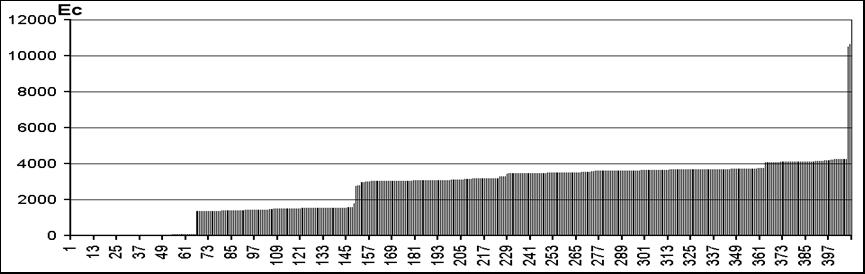Fig. 4.3.2.5. Demonstration of a real case “locked” period. Sudden steps indicate the time of occurrence of EQs (abrupt energy release, Ecx1E18=ergs).

The (Ec) was calculated for a total period of 34 years, before its occurrence, with sampling interval of one month. Two major (Ec) increase steps, plus some smaller ones, characterize the graph. The step, located, on the right end of the graph, is the one that corresponds to the seismic event, its magnitude is to be, retrospectively, predicted. Major steps indicate large amounts of energy release (strong EQs), while smaller steps indicate the occurrence of seismic events of minor importance.
Consequently, taking into account short periods of time, for the seismic data history of the area under study, only small magnitude EQs can be estimated. On the other hand, taking into account long periods of time, it is possible to identify long, corresponding “lock” periods and therefore, large magnitudes can be calculated. In the present case, the rate of cumulative energy release was calculated by using the (Ec) levels, immediately, after the two strong events (67, 151 time axis of graph). The two cumulative energy levels, just after these two major, seismic events (1969, 1976), indicate the expected Ec(t) value under continuous normal seismic energy release (Fig. 4.3.2.6).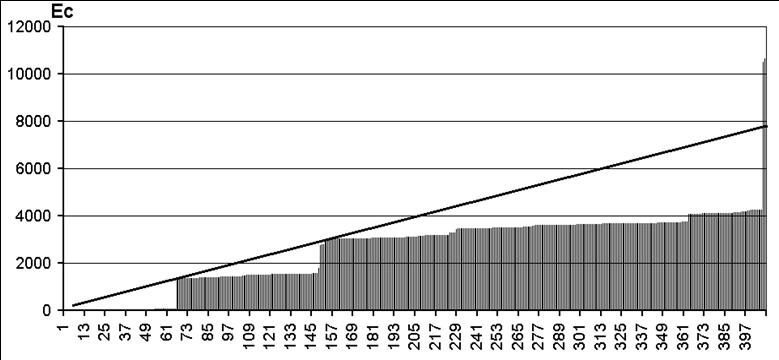Fig. 4.3.2.6. Normal, out-flow energy (Ecx1E18=ergs) release rate (solid line) determination for the period 1964 -1997.

The previous graph shows that prior to each strong EQ, a long “lock” period preceded it. The last “lock” period started on time 151, although seismic events, of minor importance, had occurred on 229 and 367 times. The latter, released some energy, but not enough, to modify drastically the last identified “lock” period. In other words, the seismogenic area was only partially, discharged. It can be said that, the area under study was “locked” finally on time 151 (1976) and from that time on, it accumulated energy which was released through the 1997 strong EQ.
In the following figure (4.3.2.7) is demonstrated the determination of the magnitude.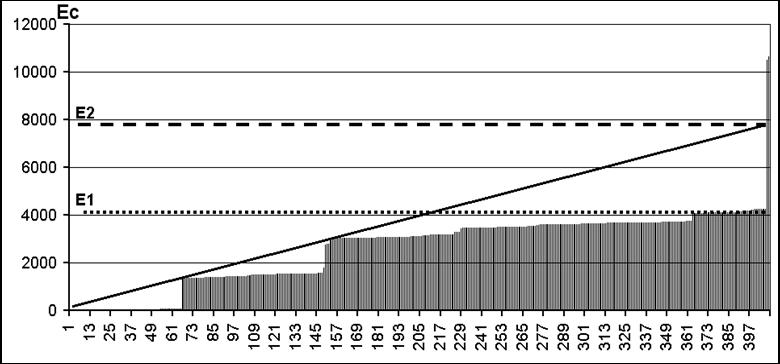Fig. 4.3.2.7. Calculation, of the expected magnitude, assuming that the origin time of the imminent EQ is known. (E1) and (E2) lines indicate the initial and final cumulative energy levels. The solid line indicates the assumed cumulative energy out-flow-rate, in time, function.

The E2 level is determined from the intersection of a vertical line on the predicted time of the expected EQ and the normal out-flow energy release rate function.
Following this procedure, the calculated magnitude for the EQ on 1997/11/18 (Ms = 6.6R) was determined as Ms = 6.5R
The magnitude Ms which is calculated by this methodology deviates for only .1 R from what was determined (Ms = 6.6R) by the Geodynamic Institute of Athens.

EQ: 18th, Nov. 1997 GEIN of Athens determination : Ms = 6.6R
Energy-flow model application :                                      Ms = 6.5R

The same procedure was applied, retrospectively, for the determination of the studied by Mizoue et al. (1978) magnitude of EQs on Kii Peninsula, Central Japan. In this work the cumulative earthquake energy release of the under study area is presented in the following figure (4.3.2.7a).Fig. 4.3.2.7a. Cumulative energy earthquake energy release graph (after Mizoue et al. 1978). Energy levels E1, E2 resulted in a Mc=4.77, while E3, E4 resulted in a Mc=4.34 for the corresponding EQs. The dashed and solid lines represent the corresponding energy release rates for both cases.

The dashed line indicates the energy flow rate for the EQ on Aug. 07, 1977, M=4.7R, while the solid line indicates the energy flow rate for the EQ on Jan 16, 1977, M=4.3. The short, solid horizontal lines (E1, 2, 3, 4) indicate the minimum and maximum, cumulative energy levels which are taken into account for the calculation of the corresponding magnitude. In the case of the EQ on Jan 16, 1977, M=4.3R, the corresponding magnitude from fig. (4.3.2.7a), is calculated as M=4.34R, while for the EQ on Aug. 07, 1977, M=4.7, is M=4.77R.
In both cases, the error in the magnitude calculation is less than 0.1R, too.4.3.3. Statistical validation test of the methodology.

A statistical test was applied to a larger data set (1972 - 2001 for EQs of Ms>=6.4R) so that the validity of the method could be verified. The data set was obtained by downloading the data file of Greek seismicity, for the period of time from 1964 to 2001, available on-line, by the Geodynamic Institute of Athens (URL address in references).
In this analysis were adopted the following parameters for the application of the postulated method:

a. Time span: The data file of the Geodynamic Institute of Athens extends (on line) back to 1964. Consequently, the seismic data were processed up to 1972 so that a minimum of 8 years past, seismic history would be available for the determination of the normal, cumulative energy out-flow rate for the subsequent strong seismic events to be studied.

b. Spatial window: It was selected as a 1.60x1.60 window, over the epicentral area to be studied, so that should be taken into account only the narrow, epicentral area, seismic history.

c. Magnitude threshold: This was selected as 6.4R so that only significantly large seismic events would be studied.

In the following Table -1 the 18 EQs, which were studied, are listed, ranking in magnitude scale. The first column indicates the year, month, day, hour, and the minute (yyyymmddhhmm format) when each event took place. The second column indicates the corresponding Latitude, the third column indicates the corresponding Longitude and the last one indicates its Magnitude in Ms (Ms=ML+.5).

TABLE - 1

 Date – time Lat. Lon. Ms 197904150619 42.00 19.00 7.3 198308061543 40.08 24.81 7.1 198201181927 39.90 24.50 6.9 198102242053 38.14 23.00 6.8 198112191410 39.20 25.30 6.8 198301171241 37.97 20.25 6.7 197205042140 35.30 23.60 6.6 199505130847 40.18 21.71 6.6 199607200000 36.11 27.52 6.6 199711181307 37.26 20.49 6.6 197806202003 40.80 23.30 6.5 198007090211 39.20 22.90 6.5 198112271739 38.90 24.90 6.5 197709112319 35.00 23.10 6.4 198102250235 38.20 23.00 6.4 198307051201 40.27 27.13 6.4 198406211043 35.36 23.31 6.4 199409011612 41.15 21.26 6.4

For each one of these EQs, the corresponding magnitude was determined, following the postulated methodology. The results are presented in the following table (2).

TABLE - 2

Observed    Determined

 Date–time Magnitude Magnitude dM 197904150619 7.3 7.25 - 0.05 198308061543 7.1 7.06 - 0.04 198201181927 6.9 - - 198102242053 6.8 6.71 +0.09 198112191410 6.8 - - 198301171241 6.7 6.73 +0.03 197205042140 6.6 6.40 - 0.20 199505130847 6.6 6.15 - 0.45 199607200000 6.6 6.51 - 0.09 199711181307 6.6 6.59 - 0.01 197806202003 6.5 6.35 - 0.15 198007090211 6.5 6.51 +0.01 198112271739 6.5 6.49 - 0.01 197709112319 6.4 6.30 - 0.10 198102250235 6.4 6.34 - 0.06 198307051201 6.4 6.31 - 0.09 198406211043 6.4 6.56 +0.16 199409011612 6.4 6.29 - 0.11

From a total of 18 strong EQs, for the 16 of them, it was possible to calculate the expected magnitude with a very high accuracy. This corresponds to an 89% success rate in magnitude calculation. For two of them the methodology failed. In the discussion, an explanation on this will be given. Further statistical processing of the dM values results in:

dM Mean = -.067R

and

dM S.Dev = 0.13R

For the 11 out of the 16 EQs, for which the magnitude determination was possible, the dM value is less than 0.1R, corresponding to a 73.33%. One only extreme value of –0.45 was calculated and the rest 4 of them were calculated with a dM value, ranging between 0.1R and 0.2R.4.3.4. Explanation of the achieved accuracy.

The accuracy, in the calculation of the magnitude for a pending, future earthquake, is surprisingly large. Whenever this methodology was discussed with seismologists, it was difficult for them to accept such a small deviation from the magnitudes, calculated, by traditional, statistical, seismological methods. It must be pointed out, too, that the predicted, seismological magnitudes deviate at least +/- 0.5 R from the real ones.
Let us assume that a seismological group calculates magnitudes of seismic events. The calculated magnitudes are affected by the error tendency of the group, due to various causes. The introduced errors on the magnitude calculations are represented by a “white noise”, superimposed, on the real magnitude values, since it is a random process.
This process is presented in the following figure (4.3.4.1).Fig. 4.3.4.1. The dependence of introduced errors (black dots), by a seismological group, in the calculation of the real magnitude value (solid horizontal line) of a seismic event is presented in sketch-drawing.

The real magnitude value, of each calculated seismic event, will correspond with the one indicated by the determined, straight line, in a least square sense, through all the individual calculations made by the same, seismological group.
If these “noisy” seismic magnitudes are used to calculate the cumulative, seismic energy release, then the following graph of figure (4.3.4.2) results. The black part of the graph corresponds to the past seismic events. In the case a future seismic event takes place, the stored, cumulative, seismic energy that will be released and which corresponds to that time of the seismic event, will be located in the extrapolated (red) part of the past (black) graph. This is presented in the following figure (4.3.4.2).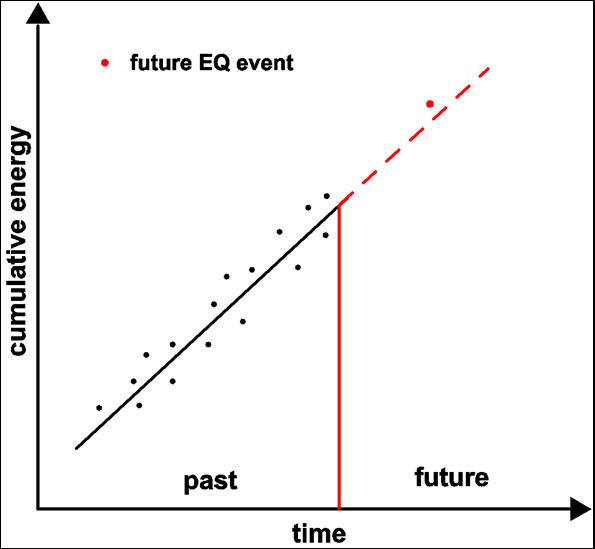Fig. 4.3.4.2. Sketch presentation of the cumulative energy that corresponds to the past (black) and future (red), seismic events.

Consequently, in case of a future earthquake (red dot) of which the time of occurrence is already known by other means, the stored, cumulative energy that corresponds to its time of occurrence, can be found by extrapolating the cumulative energy black graph of figure (4.3.4.2), in time. Therefore, working backwards for the calculation of its magnitude, inevitably, its magnitude will deviate at most, as indicates the “white noise”, which was introduced by the seismological group which made the calculations. Even better, the deviation will be less, since it will be located on the real value magnitude graph which is calculated in the least squares sense. The latter is presented in the following figure (4.3.4.3).Fig. 4.3.4.3. Correlation of the magnitude of a future, seismic event (red dot) in relation to magnitudes (black dots), calculated, by seismological methods and the errors involved in this seismological procedure.

In conclusion, the achievement of better accuracies (in the majority less than +/- 0.1 R) in predicted magnitudes, compared with the calculated ones (+/- 0.5 R at best) by statistical seismological methods, should not be considered as a surprising fact.
Actually, what can be said in simple words, about this technique is that, past seismicity constructs the cumulative energy graph in a least-square sense and this very same graph is exactly used to calculate, backwards, the exact magnitude of the future, seismic event.4.3.5. Examples of the application of the methodology on real EQs.

The “Lithospheric Seismic Energy Flow Model” methodology was tested “a posteriori” against known past strong earthquakes, whose seismic parameters of location, time of occurrence and seismological magnitude are already known. The following earthquakes were selected as typical examples:

a. Skyros, Greece, 26/7/2001, Ms = 6.1 R.

b. Northridge EQ, California, USA, 17/1/1994, M = 6.7 R.

c. Parkfield EQ, California, USA, 28/9/2004, Mw = 6.0 R.

The last two examples were chosen to make it clear that, the methodology is undependable on place and seismology research group, provided that a regular, earthquake catalog is available, concerning the past seismicity of the under study area.4.3.5.a. Skyros, Greece, 26th July 2001, Ms = 6.1 R.

The postulated methodology was applied to Skyros EQ. A few days before the occurrence of Skyros EQ, electrical precursory signals were recorded by the (VOL) monitoring site.
The location of Skyros azimuthal direction resulted as the source of their origin after detailed processing of these signals (Thanassoulas et al. 2001). Moreover, suddenly the seismic activity increased on Skyros regional area (EQ on 21st July, Ms = 5.1R took place), indicating thus, in an indirect way, the regional area that was activated seismically and consequently generated the emitted and recorded, electrical signals.
Starting with this hint, the postulated methodology was applied to a window of 1.60x1.60, centered, on the epicentral area of the 5.1R EQ on 21st July, in an attempt to predict the magnitude of the expected EQ. The timing of Skyros EQ was calculated by other methodology (Thanassoulas et al. 2001a, b). Therefore, it was a simple task to detect the presence of any seismic “lock” state of the regional seismogenic area of Skyros, by studying the past seismicity of this area, and if any, to calculate the magnitude of the oncoming EQ. The period considered for the past seismic history of the area spans from 1988 to July 22nd, 2001.
At a first approach, a magnitude of M = 6.1R was calculated. This value was based on the last “lock” period, detected, in the regional area of Skyros. The calculated magnitude equals Ms = 6.1R and fits the value, provided, by the Greek Seismological Observatory in Patras. This is shown in the following figure (4.3.5.a.1).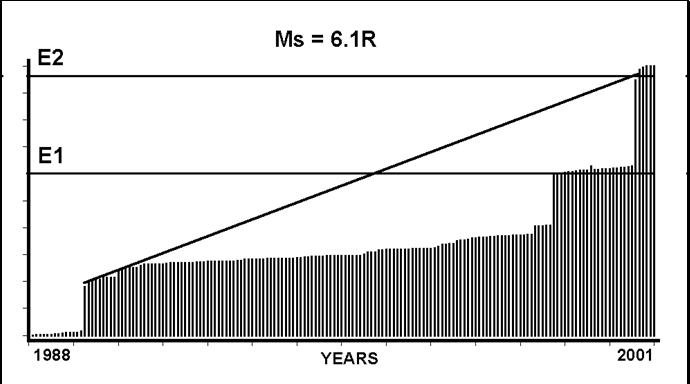Fig. 4.3.5.a.1. Magnitude determination is shown, for the last lock period.

The normal energy out-flow rate at Skyros area, is indicated by the slope of the trend (solid line) that starts just after the first seismic event on the left side of the graph. In the next figure (4.3.5.a.2) is considered a longer “lock” period. The calculated magnitude equals Ms = 6.3R and fits the value provided by the Greek Seismic Observatory in Thessaloniki.Fig. 4.3.5.a.2. Magnitude determination, when a longer lock period is considered.

Nevertheless, no matter what the actual, real magnitude of this EQ is, both solutions presented herein, are in very good agreement to the magnitude, calculated, by most seismological observatories.4.3.5.b. Northridge EQ, California, USA, 17/1/1994, M = 6.7 R

The validity of the LSEFM methodology was tested, on the strong EQ of Northridge 17th January 1994 (M=6.7R, lat = 34.21, long = -118.54), too. In the following figure (4.3.5.b.1) the epicentral area of Northridge EQ is presented by a blue circle.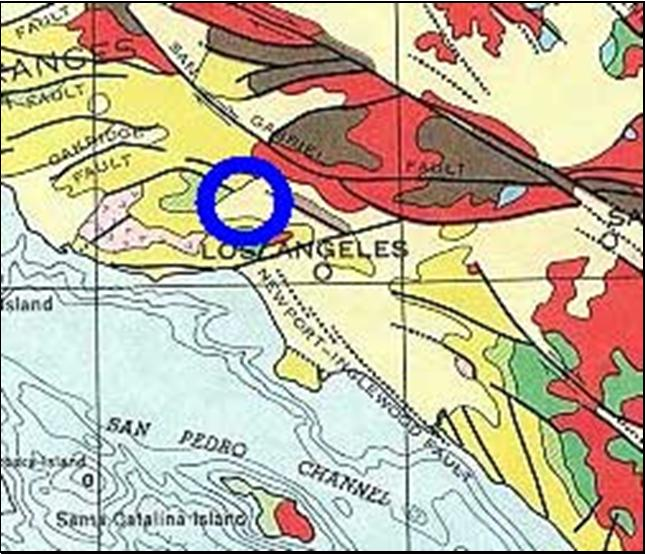Fig. 4.3.5.b.1. Location map of Northridge EQ (blue circle) and the Los Angeles city (map after USGS).

Following the methodology of the "LSEFM", the cumulative, seismic energy release graph was constructed for the period 1932 to 2004. The sampling interval is (1) month. That is each Y value of this graph represents the total, seismic energy, released, over a month. The cumulative, seismic energy release was studied over a rectangular area around Los Angeles and is indicated by the coordinates:

Upper left: 34.75/-119.25 Upper right: 34.75/-117.25,

Lower left: 33.25/-119.25 Lower right: 33.25/-117.25.

The calculated, cumulative energy release graph is presented in figure (4.3.5.b.2).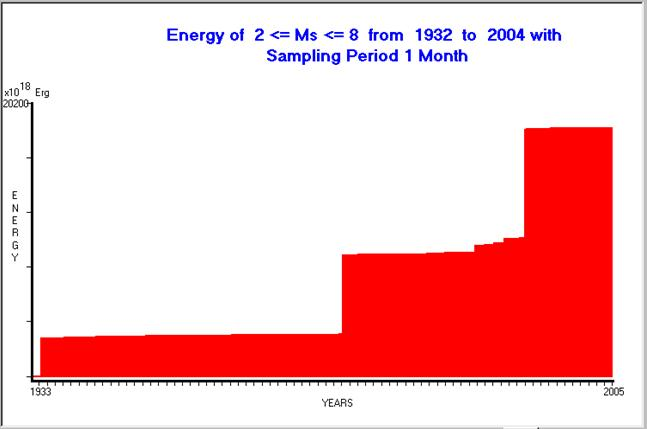Fig. 4.3.5.b.2. Cumulative, seismic energy release, for the period 1932 - 2004, calculated, for the regional area of Northridge EQ.

The abrupt steps of the graph indicate the occurrence of strong EQs (in the past), in the studied frame.
From left to right the following three EQs are shown:

a. 1933, March 11th, Lat. = 33.64 Long. = -117.97 Magnitude = 6.4R

b. 1970, February 9th, Lat. = 34.42 Long. = -118.37 Magnitude = 6.6R

c. 1994, January 17th, Lat. = 34.21 Long. = -118.54 Magnitude = 6.7R

What is obvious, from the previous figure (4.3.5.b.2), is the fact that the regional area of Northridge - Los Angeles remains in a "locked" state, for very long periods, acquiring seismic energy, through any suitable, physical, seismological mechanism, no matter what it is.
The accumulated, seismic energy is released, mainly, through strong EQs. Energy release through small-size seismicity does not modify, significantly, the appearance of the graph. The LSEFM method indicates indirectly, the "normal, cumulative energy release time-function", under unlocked conditions. Therefore, the next to come strong EQ magnitude can be calculated by taking into account the seismic history of the area under study. This is presented in the following figure (4.3.5.b.3).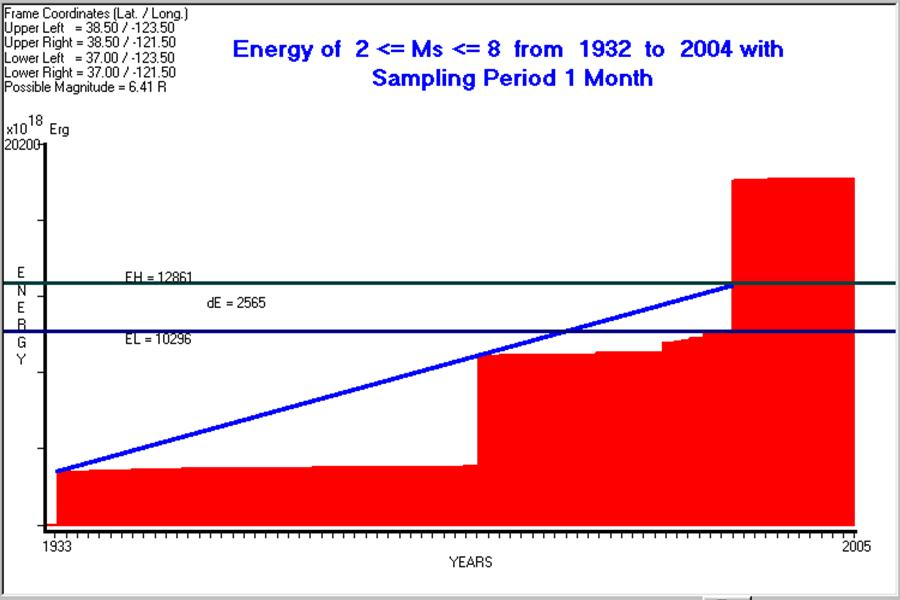Fig. 4.3.5.b.3. Calculation of magnitude of Northridge EQ is shown, by using the "LSEFM" methodology.

The seismological methods determined a magnitude for the EQ in Northridge of M = 6.7 R. The lithospheric, seismic energy flow model, which was applied to the regional area of Los Angeles, calculated its magnitude as M = 6.45 R. The normal, seismic energy flow rate is indicated by the two peaks of energy release, generated, from the two earlier, strong seismic events (1933, 1970). The difference in magnitude, between the two methods, is only dM = .29 R, not too bad at all. It must be pointed out, too, that the epicentral area and the timing of the EQ in Northridge, which were used in this test, were known in advance (a posteriori study).4.3.5.c. Parkfield EQ, California, USA, 28th September 2004, Mw = 6.0 R.

The LSEFM method was applied to the regional area of Parkfield, in an attempt to calculate the magnitude of the earthquake on September 28th, 2004, Mw=6.0R. The details are as follows:
The adopted, seismogenic area is the one presented in the following figure (4.3.5.c.1).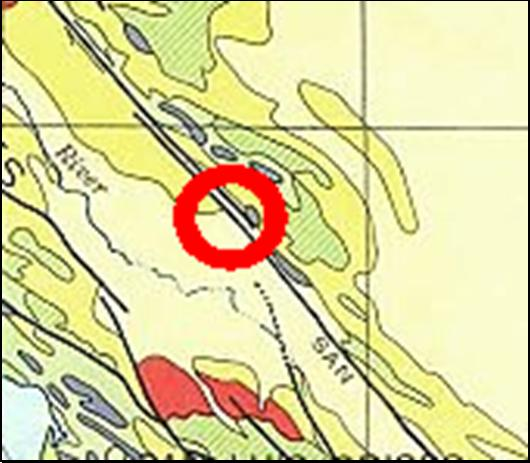Fig. 4.3.5.c.1. Location – geological map of the earthquake in Parkfield (red circle) and used seismic, energy release frame location map (map after USGS).

The coordinates of the used frame are:
Upper left: 36.6 / -121.1 Upper right: 36.6 / -119.5
Lower left: 35.0 / -121.1 Lower right: 35.0 / -119.5
The calculated cumulative seismic energy release graph vs. time is shown in the follo-wing figure (4.3.5.c.2).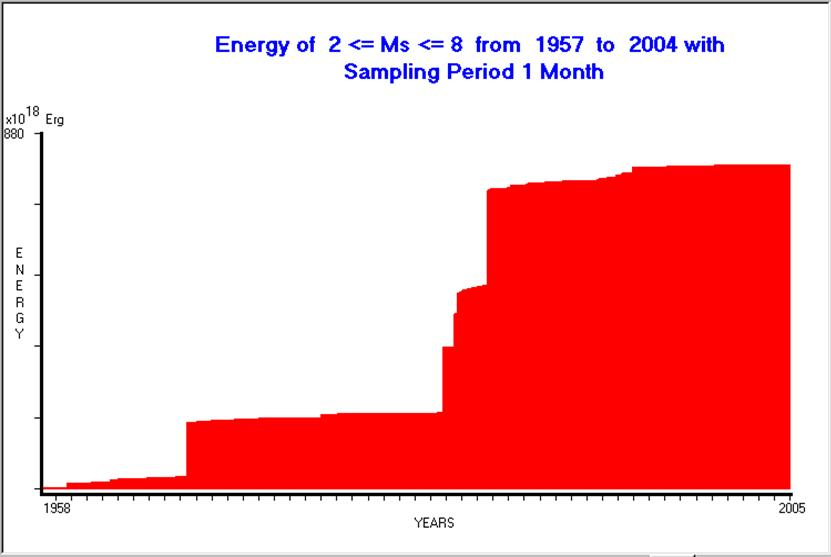Fig. 4.3.5.c.2. Calculated cumulative seismic energy release is shown, for the period 1957 - 2004, for Parkfield area.

The calculated magnitude of Parkfield earthquake is Ms=5.94R (fig. 4.3.5.c.3), compared with the Mw=6.0R, calculated, by seismological methods.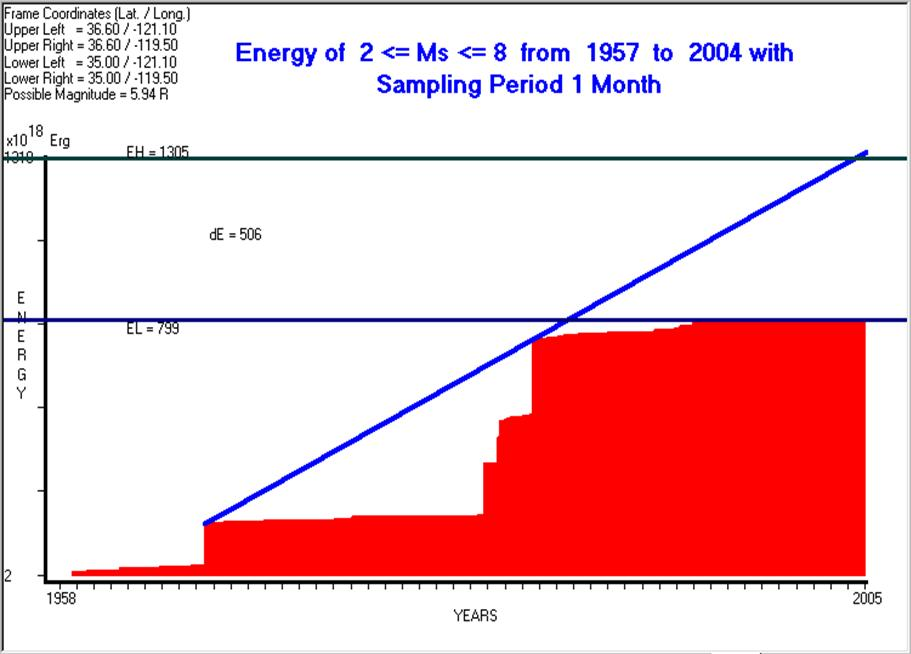Fig. 4.3.5.c.3. Calculation of the magnitude of the strong EQ, in Parkfield area, that occurred on September 28th, 2004.

4.3.6. The BIG ONE earthquake, expected, in California.

Several seismological studies and related observations indicate the probability of a strong EQ to strike, in future, in the area in Southern / Northern California. It is speculated, too, that a magnitude of 8 R could be possible (therefore, it is called as THE BIG ONE), but it is still unknown, when this event will take place. It must be pointed out that, an 8R earthquake is almost 33 times larger, in terms of released energy, than a 7R EQ and 1000 times larger than a 6R EQ. Therefore, the graph of the seismic, cumulative energy to be released, before the occurrence of the BIG ONE, must present a corresponding, high value of change, in the energy flow rate, before its occurrence.
The possibility of the BIG ONE is investigated, using the LSEFM method, for both Los Angeles / San Francisco regional areas and Southern / Northern California, as well.

4.3.6.1. Los Angeles area.

As a working hypothesis, it is assumed, in this case, that the present seismological energy flow rate status, of the regional area of Los Angeles, will not change up to year 3000. As a spatial frame, for the calculation of the cumulative energy flow was used the one in figure (4.3.5.b.1).
The cumulative energy out-flow graph was projected up to the year 3000 and the expected magnitude of the future strong EQ was calculated for specific years of occurrence. The results are presented in the following TABLE – 1

TABLE – 1

Y E A R     MAGNITUDE
(in Richter)

 2010 6.69 2020 6.82 2030 6.92 2040 6.99 2050 7.05 2100 7.23 2200 7.42 2300 7.53 2400 7.61 2500 7.68 2600 7.73 2700 7.77 2800 7.81 2900 7.84 3000 7.88

The year, when the calculation of the magnitude is made, is indicated in the first column, while in the second one is presented the calculated magnitude. Assuming that, during this period of time (almost 1000 years), no significant EQs will occur, which could release a large amount of seismic energy, then the calculated magnitudes are correct, according to the methodology that is followed. The seismological observations, of the previous history of the under study area, indicate that the latter is rather impossible, since a repetition period of 30-40 years is quite common for the strong EQs of the above area. Therefore, the calculated magnitudes have to be less than what was calculated for this period of time. In such a case, the magnitude of 8 R for the regional area of Los Angeles is rather unrealistic, even within the next 1000 years.4.3.6.2. Southern California area.

As a working hypothesis, it is assumed, that the entire Southern California is the seismogenic area under study. The following coordinates define the area under consideration:

Upper left: 36.00 / -121.00 Upper right: 36.00 / -114.5

Lower left: 32.75 / -121.00 Lower right: 32.75 / -114.5

and is presented in the following figure (4.3.6.2.1).Fig. 4.3.6.2.1. Spatial window used, for the study of the Southern California (map after USGS).

The calculated cumulative energy out-flow for this spatial window, is presented in the following figure (4.3.6.2.2).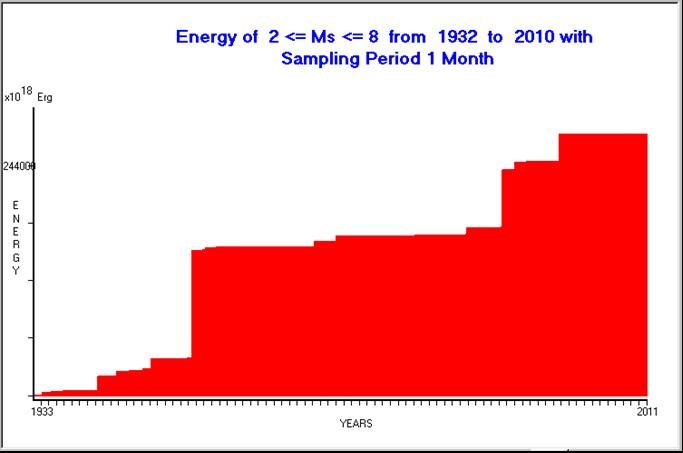Fig. 4.3.6.2.2. Cumulative, seismic energy release, for the period 1932 - 2010, calculated, for the area of Southern California.

The calculated magnitudes for the next 1000 years are presented in the following TABLE - 2.

TABLE – 2

Y E A R       MAGNITUDE
(in Richter)

 2004 7.09 2010 7.23 2020 7.38 2030 7.43 2040 7.52 2050 7.61 2100 7.75 2200 7.94 2300 8.05 2400 8.14 2500 8.19 2600 8.25 2700 8.3 2800 8.33 2900 8.37 3000 8.39

The higher values, observed, (for almost 0.5R), of Table - 2 compared with Table – 1, are attributed to the seismicity of the area, outside the regional area of Los Angeles. In any case, significant, larger values (M>8R) of magnitude above the one of the "BIG ONE" are valid, under the present, constant, seismic conditions, almost after 300 years. In case that normal, strong EQs (M=6-7R) occur during this period of time, then the magnitudes of Table - 2 will be slightly decreased and therefore, the time span for initiating an 8R EQ will increase, accordingly.4.3.6.3. San Francisco area.

In a similar way, the expected values, for a hypothetical future strong EQ, for San Francisco frame area and for each specific time of occurrence up to year 3000, were calculated and are presented in TABLE - 3.

TABLE – 3

Y E A R          MAGNITUDE
(in Richter)

 2010 6.44 2020 6.56 2030 6.63 2040 6.67 2050 6.71 2100 6.88 2200 7.06 2300 7.17 2400 7.25 2500 7.31 2600 7.36 2700 7.41 2800 7.45 2900 7.48 3000 7.51

The maximum, expected magnitude for a future, strong EQ is M = 7.51R, theoretically in year 3000.
This result is due to the fact that there is not any large, seismic energy, stored, in the frame of San Francisco area. This is indicated, too, by the study of its cumulative seismic energy release in the past, by using the available file that concerns the seismicity of the area.4.3.6.4. Northern California area.

Finally, the possibility for the "BIG ONE" is investigated for the regional area of Northern California. The used seismic energy release frame is presented in the following figure (4.3.6.4.1).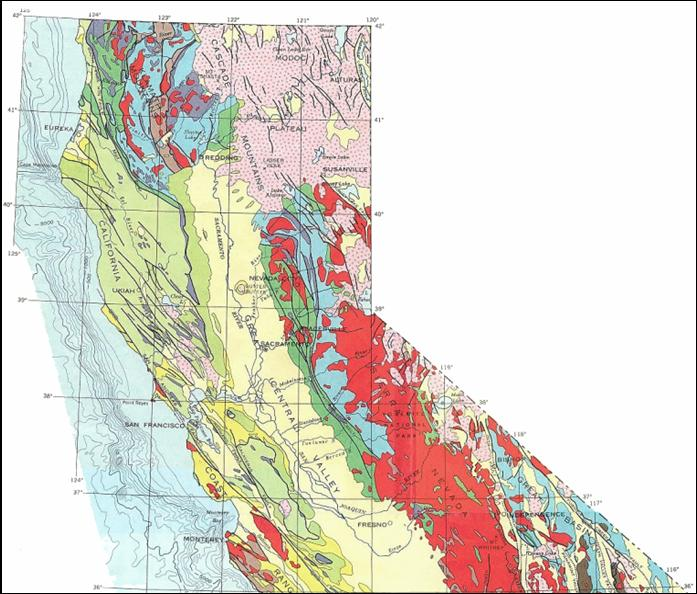Fig. 4.3.6.4.1. Spatial window (seismic energy release frame) used for the study of the Northern California (map after USGS).

The corresponding, cumulative, seismic energy release graph is presented in the following figure (4.3.6.4.2).Fig. 4.3.6.4.2. Cumulative, seismic energy release, for the period 1910 - 2010, calculated for the Northern California Area.

Following the same methodology, were calculated, and are presented in TABLE – 4, the expected magnitude values, for a hypothetical, future strong EQ, for the Northern California frame area and for each specific time of occurrence, up to year 3000.

TABLE – 4

Y E A R        MAGNITUDE
(in Richter)

 2004 7.71 2010 7.73 2020 7.79 2030 7.83 2040 7.88 2050 7.91 2100 8.04 2200 8.2 2300 8.3 2400 8.38 2500 8.43 2600 8.48 2700 8.53 2800 8.56 2900 8.6 3000 8.63

Theoretically, for an EQ of 8R magnitude, the required seismic energy could be accumulated around year 2100. The calculated larger magnitude values are due to the large extent of the frame area (Northern California) and therefore, larger seismic energy release is totally observed vs. time all over the area under study.

In short, the following concluding remarks can be made:

a. From Table - 1, it is suggested that a 7.5R EQ could hit the regional area of Los Angeles in a period of time not earlier than 300 years, provided that the seismic status does not change at all. This period can be extended more, because of the seismic events, which will probably occur within the next 300 years, of intermediate (6-7R) magnitude, which will release part of the seismic energy which is stored in the regional area.

b. From Table - 2, it is suggested that a 7.5R EQ could hit the area of Southern California in a period of time not earlier than 40 years. This period could be extended more for the same reasons as for (a).

c. From Table - 1, THE BIG ONE takes almost 1000 years for the accumulation of the required, seismic energy (M = 8R), for the case of the regional area of Los Angeles. This period of time could be prolonged more because of the reasons, presented, in paragraph (a).

d. From Table - 2, THE BIG ONE takes almost 300 years for the accumulation of the required seismic energy (M = 8R) for the case of the area of Southern California. This period of time could be prolonged more for the reasons, presented, in paragraph (a).

e. From Table - 3. Within the time span up to year 3000 AC, the maximum, expected, EQ magnitude is M = 7.51R for the case of the area of San Francisco.

f. From Table - 4. THE BIG ONE takes almost 100 years for the accumulation of the required seismic energy (M = 8R) for the case of the area of Northern California. This period of time could be prolonged more because of the reasons presented in paragraph (a).

The following two questions are of great interest:

Which is the most probable area, in Southern / Northern California, to be hit in the future by a strong EQ?

This question can be answered by the construction of the seismic, potential map (see following presentation) of Southern / Northern California. This map will present the seismic energy accumulation spatial distribution. Its maximum values indicate the most dangerous areas to be hit in the future.

When will a strong EQ (or the BIG ONE) hit?

Strong EQs are preceded very often by precursory, electrical signals which are generated at the focal area and depend, quite well, on the tidal waves of the lithosphere. These signals are kind of short-term warning that an EQ is initiated and the focal area is at a state just before collapsing. Therefore, these signals not only signify the short-term time window which is needed for the future strong EQ prediction, but they provide the means for its epicentral area determination, too.
Finally, what is necessary, for the utilization of an effective monitoring of an oncoming strong EQ, is the installation of a network for monitoring the EQ generated, precursory, electrical, signals, all over the area of Southern / Northern California. An example of such a network, the installation of which is still in progress, over Greece, is presented later on.4.3.7. Some different seismological applications of the “Lithospheric Seismic Energy Flow Model - LSEFM”.

The LSEFM model, not only provides a solution for the prediction of the magnitude of a future, strong EQ, but even more, it presents a physical justification for the “accelerating, seismic energy release - deformation” and “seismic quiescence”, which is frequently observed before many strong EQs.
Moreover, starting from this model, it is possible to calculate maps of the seismic energy which is stored in large seismogenic areas (seismic potential maps). Therefore, it is possible to use them as a tool for the intermediate term characterization of seismic prone areas. An example of such, generated, maps, is presented for the Greek territory.
Both applications are presented as follows:4.3.7.1. Accelerated, seismic energy release and deformation.

The rate of seismic energy release, as a function of an inverse power of the time remaining to the main seismic event, was proposed and applied to back-analyses of several foreshock main sequences by Varnes (1987a, b).
In these papers, Varnes analyses the accelerating precursory, seismic activity, in terms of seismic moment release. A simple in time (t), empirical power law failure function was postulated (Bufe and Varnes, 1993) that relates the parameters of the remaining time (tc-t) to the occurrence of the imminent strong EQ and its corresponding magnitude (M) to the seismic moment release (the term, accumulated Benioff strain, is often used). Main (1995) studies the earthquakes from the point of view of a critical phenomenon.
In the same area of Statistical Physics (Main, 1996), Bowman et al. (1998) showed that the cumulative, seismic strain release increases as a power law time to failure before the final event. Papazachos et al. (2000) used the same methodology to study the Benioff strain rate release of the Aegean area. Moreover an estimate for the timing of an imminent strong EQ was achieved, with an accuracy of +/- 1.5 years, by using the same methodology for EQs of the Aegean area (Papazachos et al. 2001). The time to failure model was used as a technique, in which a failure function fits on a time series of accumulated Benioff strain, by Di Giovambattista and Tyupkin (2001) in order to analyse the relation of the time-to-failure model to the hypothesis of fractal structure of seismicity. This method was applied to in laboratory rock samples fracturing and real strong EQs of Kamchatka and Italy, as well.
In the early papers, which deal with the “Benioff strain - accelerated deformation – critical point” method, the seismogenic, studied area was related to a circle of an optimum radius R and, lately, to an elliptical area (Papazachos et al. 2001).
The common feature, of all these papers, is the absence of a physical model that accounts for the time to failure well-known equation which is used very often. This equation is an empirical one, and derives from the Statistical Physics applied to other fields of Applied Science. Moreover, no tectonic information is taken into account, as far as it concerns the seismogenic under study area.
The topic of accelerating deformation is faced from another point of view and it is analyzed by the use of the “Lithospheric, Seismic Energy Flow Model” which was presented in section (2.5.1.1).
In this analysis is introduced an important new feature, that, of the deep, lithospheric, fracture zones (Thanassoulas, 1998). These zones are the main, seismogenic zones where strong EQs occur and constrain the extent of the seismogenic areas to be studied.
If we recall the theoretical analysis which was already presented and summarizing the forms the equation Ecum(t) takes (that represents the energy flow) the following cases are possible:

a. linear polynomial - constant energy flow

b. higher order polynomial - real acceleration

c. accelerated for a time period, long before the main seismic event, which is followed by a constant energy flow period, just before the occurrence of the large EQ.

Cases (b) and (c) are represented very often by the well-known “time to failure” function.
Although, mathematically, it is possible to transform any polynomial to any arbitrary function, i.e. time to failure function, by calculating the appropriate parameters of the latter, by using LSQ techniques, still remains the parameter of arbitrarity, as far as it concerns the validity of physics behind this transformation.
Moreover, the time to failure function depends on two variables. The first one is the magnitude of the imminent EQ and the second one is the time to failure left. In order to overcome the problem of solving a two parametric equation (infinite number of solutions), the parameter C (Bowman et al. 1998) was introduced, that is the ratio of power low fit error over the linear fit error, as far as it concerns the cumulative seismic energy release. Still the notion of this ratio is totally arbitrarily, set.
Therefore, it is suggested that the magnitude and time to failure, of a strong imminent EQ, calculated by these methodologies, are not supported by any validated physical mechanism and should be rejected.
The validity of the LSEFM model application on seismic accelerating deformation and energy release phenomena is demonstrated by the following two examples. The first one refers to Zakynthos, Greece 2002, Ms = 5.8R EQ and the second one refers to an area (called A) that is in accelerating mode but the EQ has not taken place yet up to 2004.
The data, which are used in this analysis, are taken from the EQ files released on-line at the web site of the National Observatory of Athens (NOA), Greece (www.gein.noa.gr).
The released cumulative energy was calculated by transforming all the EQs, of the area under study, into the corresponding energy release, for each seismic event, regardless of their magnitude.Zakynthos EQ example.

The postulated methodology was applied over the regional area of Zakynthos, indicated by a red circle in figure (4.3.7.1.1). In this area, was observed large accelerated seismic energy release during the second half of 2002. This ended in a strong (M=5.8R) seismic event on 3rd December 2002.Fig. 4.3.7.1.1. Zakynthos regional (red circle), seismic area is presented.

By taking into account the deep lithospheric fracture zones (Thanassoulas, 1998) that control the seismicity of the regional area, a trapezoidal area (fig. 4.3.7.1.2), which surrounds the main tectonic features of Zakynthos area, was selected. It is assumed that, the energy release, in this area, is due to seismic activity that occurs in these lithospheric, fracture zones.Fig. 4.3.7.1.2. The blue frame indicates the area whose seismic energy release is studied. The brown line indicates the main lithospheric, deep fracture seismic zone.

The released energy (fig. 4.3.7.1.3) was calculated for the period between 2000 and end of November 2002. The sampling interval, which was used, is 10 days. That is each 10 days the released energy was summed up to form a data point of the cumulative energy graph. It is worth to note that the accelerating process was identified well, before the Zakynthos seismic event.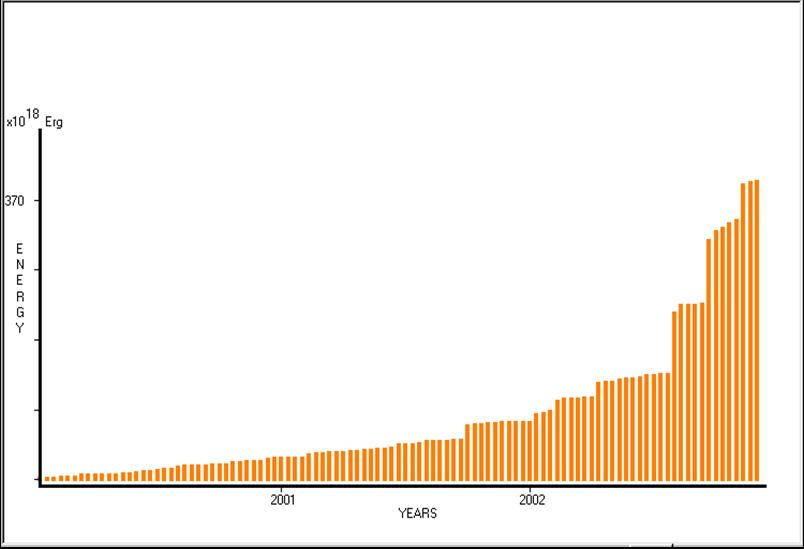Fig. 4.3.7.1.3. Accelerated, cumulative, seismic energy release that corresponds to the period 2000 – 2002, for the regional (frame) area of Zakynthos. Sample interval = 10 days, is presented.

The data presented, in the previous graph (fig. 4.3.7.1.3) were fitted with a 6th degree polynomial. This is demonstrated in the following figure (4.3.7.1.4).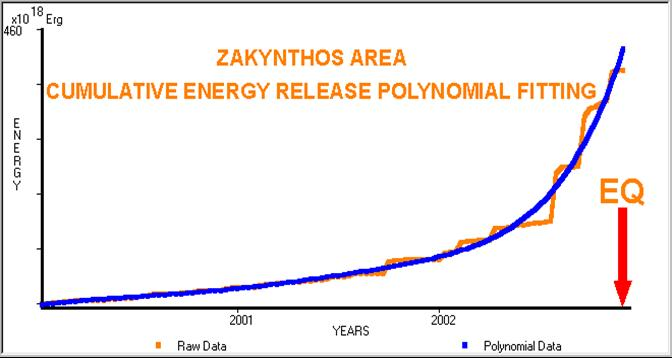Fig. 4.3.7.1.4. Calculated cumulative energy release data for the area of Zakynthos (brown line) fitted with a 6th degree polynomial (blue line). The red arrow indicates when the strong, EQ occurred.

A different point of view of the accelerating energy release is through the study of its time-gradient. This is demonstrated in the following figure (4.3.7.1.5).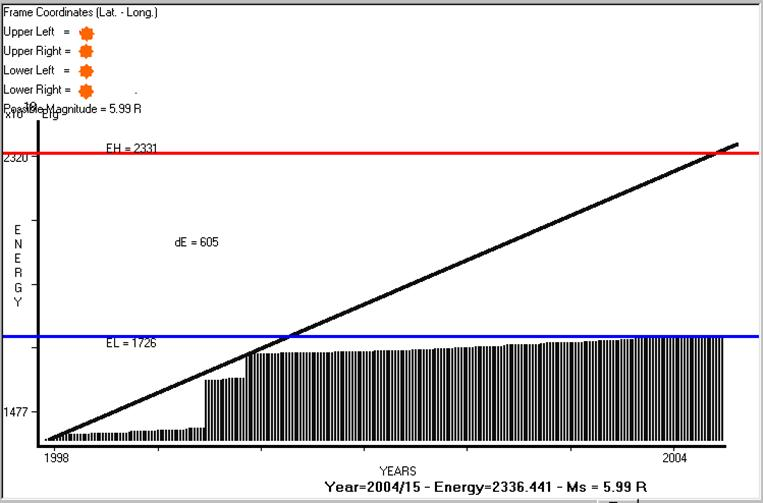Fig. 4.3.7.1.5. Time gradient (year 2000 – 2002) of the cumulative energy release of the area of Zakynthos is presented.

In figure (4.3.7.1.5), the time-gradient was calculated, analytically, after having obtained the polynomial function of cumulative energy release, presented in figure (4.3.7.1.6).Fig. 4.3.7.1.6. The analytical presentation of the polynomial function that represents the cumulative energy release of the area of Zakynthos for the period from 2000 to the end of 2002 is shown.

Finally, in figure (4.3.7.1.7), is shown, the location of the area of Zakynthos EQ in relation to the deep, lithospheric, seismic fracture zones.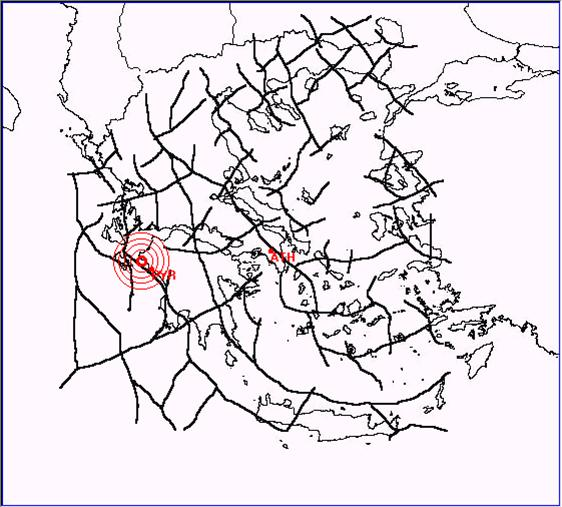Fig. 4.3.7.1.7. Location of the area of Zakynthos EQ, Ms = 5.8R, 3/12/2002

The close coincidence of the EQ epicentral location with the adjacent, deep, lithospheric fracture zone justifies the presence of the latter, as this was demonstrated by Thanassoulas (1998).
It was stated, earlier, that a decelerating seismic energy release period of time preceded the last phase of the preparation of a strong EQ. This last phase of decreased, seismic energy release is what the seismologists call as a “seismic quiescence”. An example of such an energy release (accelerating deformation followed by a seismic quiescence) sequential case was presented by Varnes (1989). In the following figure (4.3.7.1.8), the accelerating seismic energy release period of time is more than evident, while at the end of the graph, the “seismic quiescence” period that preceded Cremasta, Greece EQ is clearly shown.Fig. 4.3.7.1.8. The “accelerating, seismic energy release” and “seismic quiescence”, observed, at Cremasta, Greece EQ, are shown (after Varnes, 1989).Seismogenic area (A) example.

Another example for the very same mechanism, which is still in progress, at a seismogenic area (called A, without location presentation at present, for preventing social implications), is presented as follows. In the following figure (4.3.7.1.9) is presented the cumulative, seismic energy release.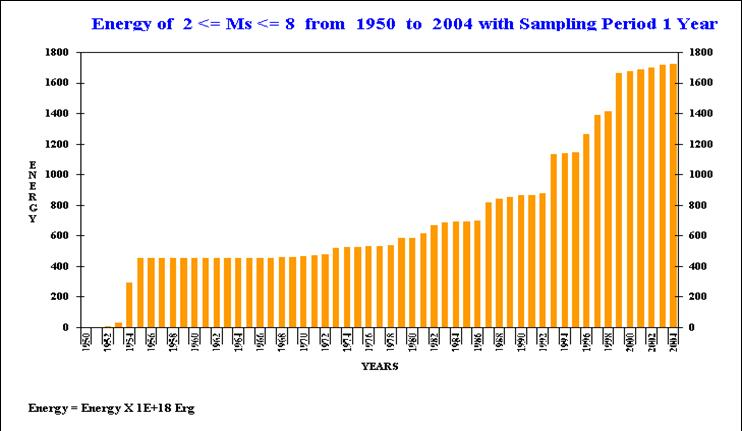Fig. 4.3.7.1.9. Cumulative, seismic energy release calculated, for seismic area (A).

The sampling interval is that of a year and the time spans from 1950 to 2004. In this graph three distinct areas can be observed.
The first one extends from 1950 up to 1955 when two steps of abrupt energy release are observed (1954 – 1955).
The second extends from 1955 up to 1999. Apart from some steps of seismic energy release (EQs), observed, the main feature of this time period is in the accelerating mode.
The last part of it that extends from 1999 up to 2004 is, clearly still, in the “seismic quiescence” mode.
The graph of figure (4.3.7.1.9) is analyzed, in more details, in the following figures. In figure (4.3.7.1.10) the released cumulative, seismic energy, for the time period 1955 – 2000, was fitted by a 6th degree polynomial.Fig. 4.3.7.1.10. Accelerating, seismic energy release, fitted by a 6th degree polynomial, calculated for the period 1995 – 2000. The blue line corresponds to the original data, while the red one corresponds to the fitted polynomial.

Although, the polynomial form indicates that acceleration of energy release is in progress, the calculation of the corresponding rate of energy release, in time, is an evaluation of the specific process at any time.
This is demonstrated in the following figure (4.3.7.1.11). The analytical derivative in time, of the calculated polynomial, was determined for the same time period.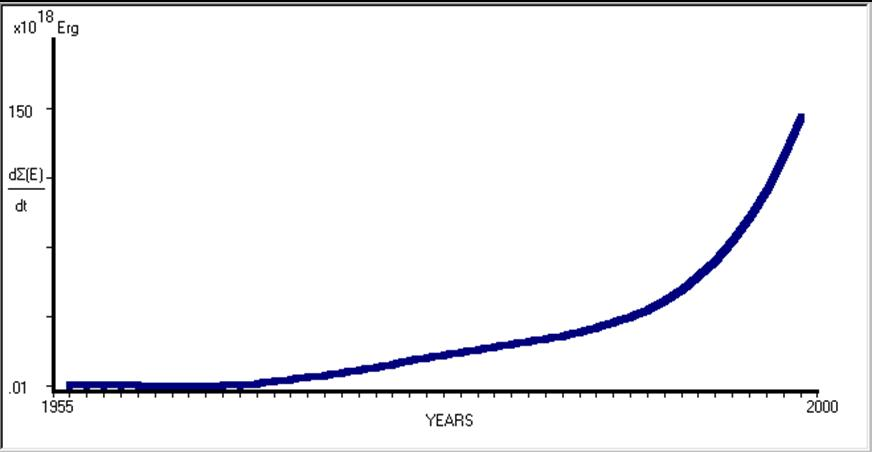Fig. 4.3.7.1.11. Evaluation of the cumulative, seismic energy flow-rate is presented, for the same period of time, 1995 – 2000.

The calculated value for the rate of cumulative energy flow (dEcum/dt) through the seismogenic area and for the time t=2000y is:

dEcum/dt = 150*1018 erg (t = 2000y).                                         (4.3.7.1.1)

The seismic quiescence period (2000 – 2004), of the graph in figure (4.3.7.1.9), is enlarged and presented in the following figure (4.3.7.1.12).Fig. 4.3.7.1.12. The “seismic quiescence” period from 2001 to 2004 is presented. Cumulative seismic energy release graph vs. time.

The analytical derivative, in time, of the calculated polynomial was determined for the same period of time. A 1st degree polynomial was fitted in this case. The results of this procedure are shown in the next figure (4.3.7.1.13).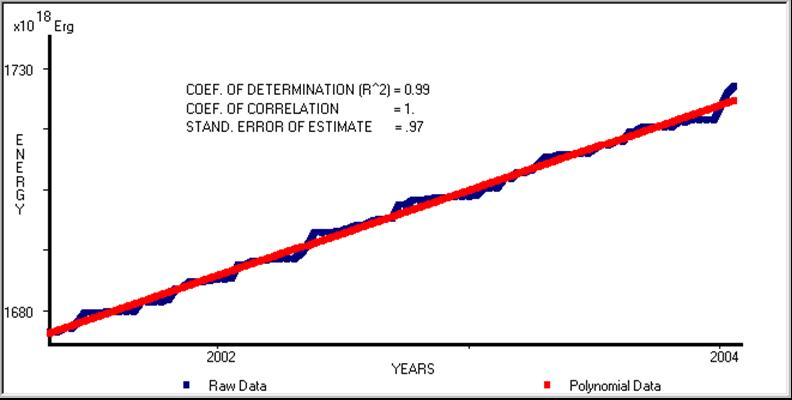Fig. 4.3.7.1.13. The analytical derivative in time of the calculated polynomial was determined for the same period from 2001 to 2004. The blue line corresponds to the original data, while the red one corresponds to the fitted polynomial.

The analytical derivative, in time of the calculated polynomial, was determined for the same period of time and is shown in figure (4.3.7.1.14).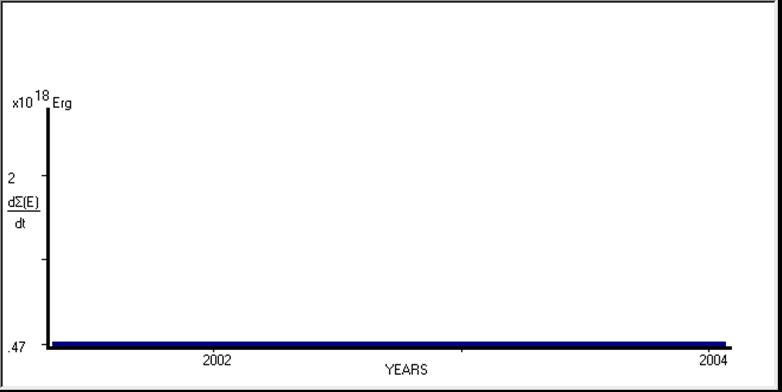Fig. 4.3.7.1.14. The analytical derivative, in time, is presented, of the calculated 1st degree polynomial for the same period of time (2001 – 2004).

The calculated value for the rate of cumulative energy flow (dEcum/dt) through the seismogenic area and for the specific period of time is:

dEcum/dt = 0.47*1018 erg (ty= 2001- 2004).                               (4.3.7.1.2)

A comparison between equations (4.3.7.1.1) and (4.3.7.1.2) shows that the cumulative seismic energy flow rate, through the under study seismogenic area, was decreased about 300 times, during the “seismic quiescence” period of time. This indicates that the seismogenic area is under mechanical “locked” and “strain charge” conditions and therefore, some time in the near future the stored, strain energy will be released through a strong seismic event. Its magnitude can be calculated by the application of the “Lithospheric, Seismic Energy Flow Model” (Thanassoulas and Klentos, 2001). The results are shown in the next figure (4.3.7.1.15).Fig. 4.3.7.1.15. Magnitude calculation of the expected, seismic event is shown. The black line indicates the normal, seismic, cumulative energy release, the blue line indicates the current level of the cumulative energy release, while the red line indicates the theoretical cumulative seismic energy release under normal conditions for time t=150 days, elapsed, in year 2004. The calculated magnitude for this time of occurrence is Ms = 5.99 R.

If the time (teq), when the seismic event occurs, is set to teq = 150 days from the start of 2004, then the seismic event will occur in a magnitude of Ms = 5.99 R.
Summarizing what was already presented it can be said that the mechanical processes that take place in any seismogenic area, mainly, control the balance of the energy flow through it. Consequently, the study of the seismic energy flow, through a seismogenic area, is capable to provide the necessary time functions that will describe what was observed in nature and was studied by statistical methods to date.
The physical mechanism of the “lithospheric energy flow model”, introduced by Thanassoulas and Klentos (2001), justifies the “accelerated deformation”, the “seismic quiescence” methods used to date and the calculation of the expected magnitude of the seismic event that will take place in a seismically, activated, area.
In contrast to the statistical / empirical “time to failure” equation, which is used by other researchers for studying the accelerated, seismic energy release, the model of the lithospheric, seismic energy flow and its mathematical analysis results, according to the specific mechanical conditions met in the seismogenic area, leads, in a mathematical way, directly to the models used to date empirically.
The mathematical analysis of the lithospheric, energy flow model does not justify, neither the use of the parameter t-teq (the remaining time to the event), nor the parameter M (magnitude). These parameters are deliberately included in the “time to failure” functions, used to date, without any physical justification. These parameters are calculated by other methodologies. Therefore, the real value of this analysis is indicative for the seismic activation of a seismogenic area that will end up with a strong event.
Quite often, a seismic quiescence (linear polynomial energy release) period of a seismogenic area is preceded by a larger period of accelerated (higher order polynomial energy release) deformation. This is the case of the last example of area A, while the same was observed in the Cremasta, Greece case (Varnes, 1989). The combined use of both metho-dologies increases the degree of certainty that a strong EQ will occur at the under study seismogenic area.
Since the methodology depends on the sampling interval of the released energy, fine details, as far as it concerns the seismic status of the seismogenic area to be studied, can be investigated by choosing a finer sampling interval.
Finally, the physical mechanism of the lithospheric, seismic energy flow model behaves in a fractal way, as it is shown from the two (2) presented examples. At the first model (area of Zakynthos), the accelerated deformation had, fully, developed within three (3) years, while at area A, the second example, this took almost 45 years.
A strong EQ, of a magnitude of M = 5.99 R, is expected to occur in area A, if this seismic event takes place up to the 150th day from the start of 2004 (around May, 2004)4.3.7.2. The “Seismic potential map”. Its application example in Greece.

The terms “seismic hazard” and “seismic risk”, are very often, referred to in the seismolo-gical and engineering geology studies.
The term “seismic hazard”, at any place, refers to quantity (H), its magnitude being the expected intensity of the ground motion at this place. The latter, can be expressed as (Papazachos et al 1985, 1989, Tselentis 1997) the expected ground acceleration, ground velocity, ground dislocation and the expected, macroseismic intensity (l).
The term “seismic risk” (R) refers to the expected results (damages in buildings, deaths etc) from the occurrence of an earthquake and depends strongly on the seismic hazard of the same place. The term (R) of the seismic risk can be expressed as the convolution of the seismic hazard (H) to the vulnerability (V) of a technical construction. Therefore, the following equation holds:

R = H * V                                                                   (4.3.7.2.1)

Tselentis (1997) presents the following, holding equation for the seismic risk:

R = H(e, μ, s)*T                                                             (4.3.7.2.2)

Where, (R) is the seismic risk, (H) is a non-linear parametric (e, μ, s) equation with (e) being the earthquake source parameters, (μ) is the propagating elastic waves media, (s) is the local conditions and (T) is the vulnerability of the technical constructions.
A seismic risk study, at a certain place, has a strong probabilistic – stochastic character and therefore all parameters that can contribute to an excess ground motion at a probabilistic level, are taken into account.
The results of a seismic risk study are presented in various forms. Probabilistic graphs vs. Mercalli scale, excess of spectral velocity and maps of spatial distribution of expected ground velocity are some of them.
A typical, seismic risk study of a place includes (Tselentis, 1997) the four following basic steps:

- Identification of the near-by earthquake sources

- Determination of the statistical model that prescribes the earthquake sources and the expected, maximum magnitude due to each one of them.

- Determination of the best amplitude decay of the seismic waves of each seismic source.

- Determination of the probability for non-exceeding any ground motion parameter level.

Maps of the spatial distribution of seismic hazard of Greece were presented in the past (Makropoulos et al. 1985, Papazachos et al. 1985, 1989). Furthermore, the Greek territory was divided in four (I, II, III, IV) zones of different expected ground acceleration, as a function of the recurrence mean time value, and the intensity (I) of a future, seismic event. The former seismic hazard map of Greece is presented in the following figure (4.3.7.2.1).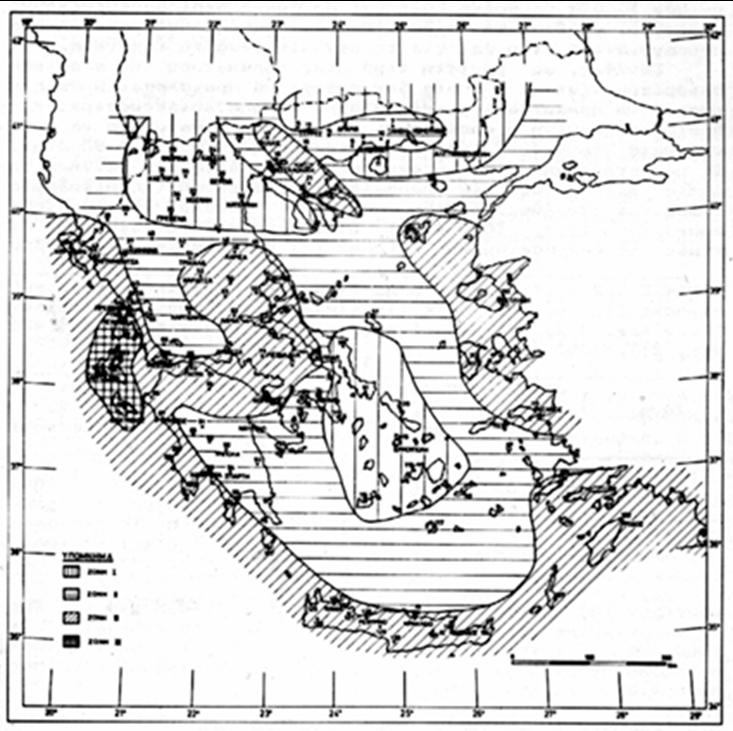Fig. 4.3.7.2.1. Former, seismic hazard zoning is presented of the Greek territory (Papazachos et al. 1989, OASP).

This map is now under revision, due to the large seismic events that took place in Greece, during the last decade (1990 – 2000).
Following the mathematical analysis which is presented by Papazachos et al. (1989), it is made clear that the seismic hazard map of Greece is based mainly on probabilistic seismic data, as far as it concerns the parameters of the earthquake sources.
The latter was made clear, when Kozani earthquake (6.6R, 13/05/1995) and Athens earthquake (5.9R, 7/9/1989) took place at areas, which were considered before as, more or less, aseismic.
Therefore, that map should be modified, as soon as possible, whenever new, seismic data are available from earthquakes that have already occurred, or even better, it should be modified according to already known data, from areas that are being highly charged with strain energy and consequently strong earthquakes are expected to occur, within some period of time (a few years).
In the recent decade, a seismological research trend was developed towards earthquake prediction (medium term prediction) by the use of accelerating seismic energy release or accelerated deformation, as it is referred very often. Main (1995), studied the earthquakes from the point of view of a critical phenomenon. Varnes (1987a, b, 1989), related the released, seismic energy to earthquake foreshock sequences in an attempt to predict earthquakes by analyzing accelerating, precursory seismic activity. A simple, in time (t), empirical power law failure function was postulated by Bufe and Varnes, (1993) that relates the parameters of the remaining time (tc-t) to the occurrence of the imminent strong EQ and its corresponding magnitude (M) to the seismic moment release. In the same area of Statistical Physics Main (1996) showed that the cumulative, seismic strain release increases as a power law time to failure before the final event. Bowman et al. (1998) used the concept of cumulative, seismic strain release, increasing, as a power law time to failure, before the final event. Moreover it was found that the critical region of radius (R) and the magnitude of the final event (M) are correlated as Log(R) = 0.5M suggesting that the strongest probable event, in a given region, scales with the size of the regional fault network.
Papazachos et al. (2000, 2001, 2002), applied the same methodology to the Aegean area, Greece. In this case, ellipses were taken into account for the critical regions, which correspond to circles of radius (R) with equal area. Di Giovambatista et al. (2001) studied the accumulated Benioff strain before strong earthquakes, by using the time-to-failure model, and presented examples from strong earthquakes that occurred at the Kamchatka and in Italy. Tzanis et al. (2003), related the crustal deformation in SW Hellenic ARC to distributed power-law seismicity changes.
Following this methodology, it is obvious, theoretically, that areas of increased probability, for the occurrence of a strong EQ, can be identified in advance and therefore, the expected, maximum magnitude of an imminent EQ, at any place, will affect, accordingly, the seismic hazard, calculated, for it.
Such maps, prepared for the entire Greek territory, will modify, accordingly, the seismic hazard map, proposed by the seismologists, and are, already, in use by the state authorities.
The above methodology has a main drawback. The acceleration of seismic energy release is not a universal process that is observed before all strong earthquakes. The application of the time-to-failure model, for the estimation of the magnitude and moment of the future, strong earthquake, is possible only in case when a tendency to acceleration is observed in the release of seismic energy in the vicinity of its epicenter. Moreover, it does not distinguish between the earthquake swarm and the foreshock activation.
This work presents a different approach in preparing such maps. These maps can be compiled, in particular, by the application, all over the Greek territory, of the energy flow model of the lithosphere (Thanassoulas et al. 2001) on the past seismic data.A. The data.

The used seismic data were downloaded from the National Observatory of Athens, Institute of Geodynamics, (NOA), Greece.
Greece is, initially, considered as a unit critical region and the lithospheric energy flow model was applied over it.
The cumulative energy (Ec) vs. time graph (fig. 4.3.7.2.2), for the period 1950 – 2002, reveals the presence of several (A, B, C) linear parts in this graph, with different energy flow rate values (EFLs). These linear parts are intermitted by periods (1, 2, 3, 4) of intense, seismic activity.Fig. 4.3.7.2.2. Greece’s cumulative energy release is presented for the time period 1950 – 2002.

The continuous decrease of the EFL values (energy flow rate), which is observed from 1950 to 2002, indicates that for the last 52 years, Greece, is charging continuously with seismic strain energy that will be released some time in the future, at some seismically prone areas.
Consequently, two questions must be answered:

- Which areas have been charged, in excess, with seismic strain?

- What is the expected maximum value of a future earthquake, for the next i.e. five years period, for each area?

The following procedure was followed, in order to answer these questions.B. Application of the method – examples.

The lithospheric energy flow model was applied over the Greek territory, by using a grid shell of 100 x 100Km. That shell slides all over Greece, in steps of 50Km from West to East and from North to South. As a result of this operation, an overlapping of 50% was applied on the obtained results and a final grid of 50 x 50Km shell was resulted.
This procedure is presented in the following figure (4.3.7.2.3).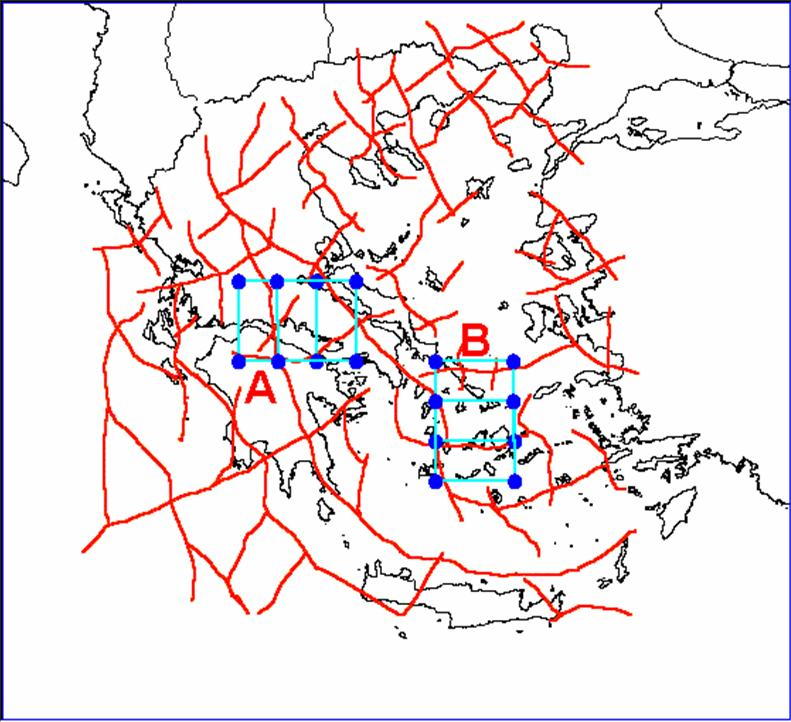Fig. 4.3.7.2.3. Size of grid shell is shown and its movement over the Greek territory. A = shell movement from West to East. B = shell movement from North to South. Both movements are performed at steps of 50Km.

For each shell position and for the defined area, the corresponding, cumulative energy versus time function, for the time period 1950 - 2000 was calculated. Determinations were made for the seismic energy strain charge potential, in terms of expected magnitude, for a future earthquake, by using this graph and following the lithospheric energy flow model procedure. As a time basis for these calculations were used the years 1970, 1975, 1980, 1985, 1990, 1995, 2000, while the expected magnitude was calculated for the next five years. For example, the calculation for the year 1980 indicates the maximum, expected magnitude for a strong earthquake in the period of 1980 – 1985.
The used shells total to a number of (323) and the obtained magnitudes for the different time periods were used to compile the corresponding maps. These maps present the strain charge status spatial distribution all over Greece, in terms of magnitude of a potential, future (within the next five years from the date of the map) earthquake.
Following, are the compiled maps for the corresponding periods of time. These maps are presented in two forms:

- The first one presents the spatial distribution of the entire range of the expected magnitude of a possible, future earthquake over the Greek territory.

- The second presents the same results, as above, but with a threshold low-level of magnitude set at 6 R. This facilitates to identify more easily the areas which are highly strain charged and therefore, prone to intense, seismic activity.

In each map, a chromatic bar indicates the corresponding earthquake magnitudes in Richter scale. The compiled maps are presented in the next pages as follows: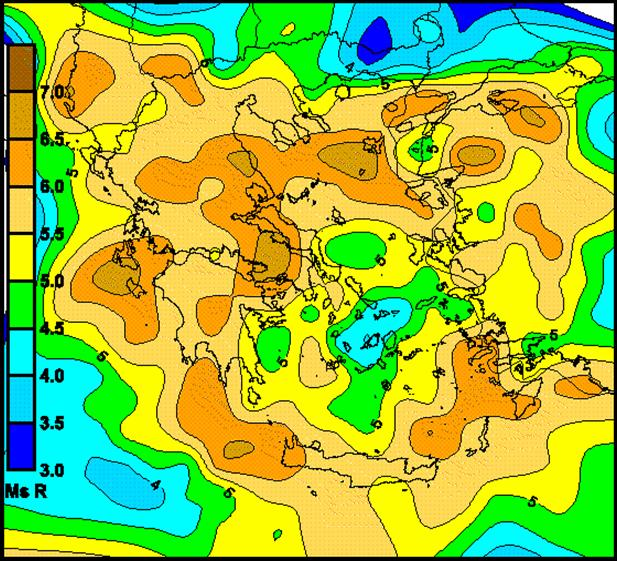Fig. 4.3.7.2.4. Calculated map, based, on data from 1950 to 1970 and expected potential earthquake magnitudes up to year 1975.

Year 1970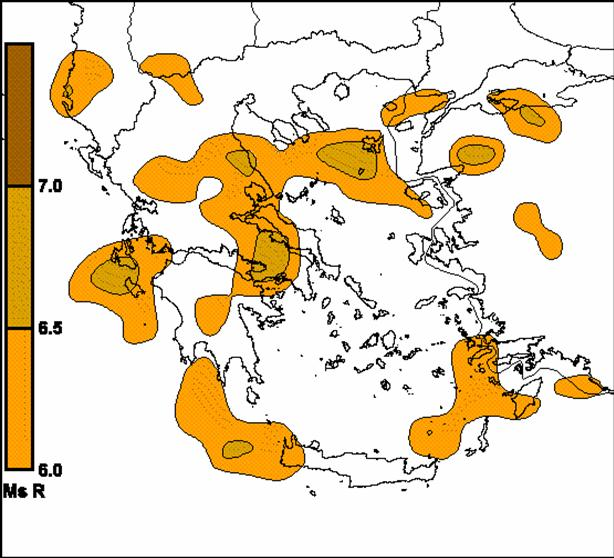Fig. 4.3.7.2.5. The same as above but a threshold of 6R was used.

Year 1975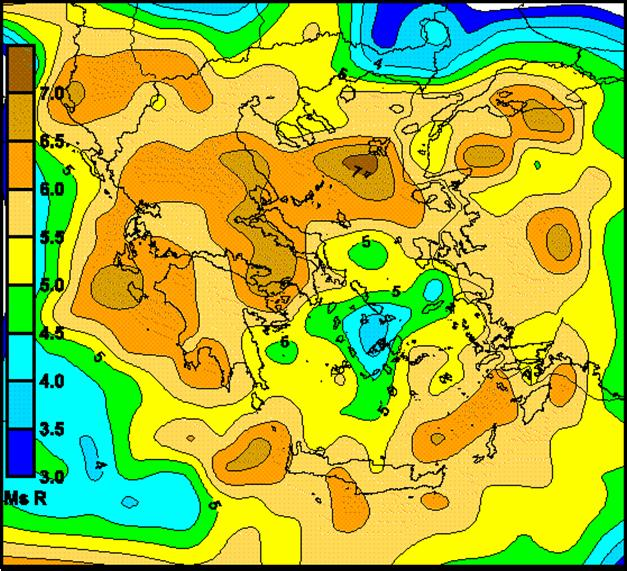Fig. 4.3.7.2.6. Calculated map, based on data from 1950 to 1975 and expected, potential earthquake magnitudes up to year 1980.

Year 1975Fig. 4.3.7.2.7a. The same as above but a threshold of 6R was used.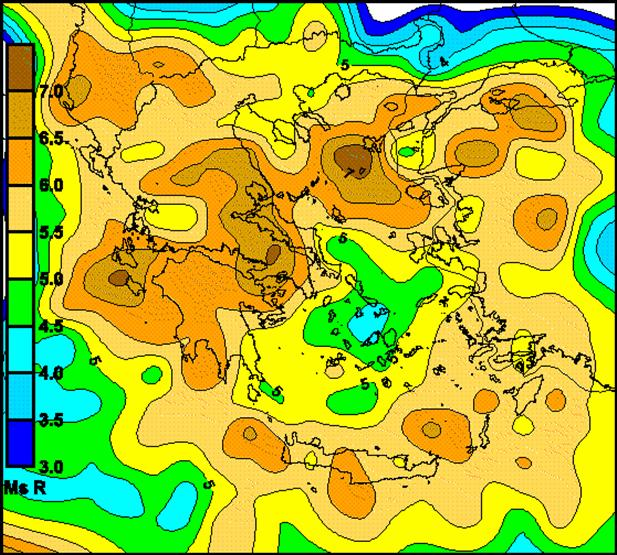Fig. 4.3.7.2.8. Calculated map, based on data from 1950 to 1980 and expected, potential earthquake magnitudes up to year 1985.

Year 1980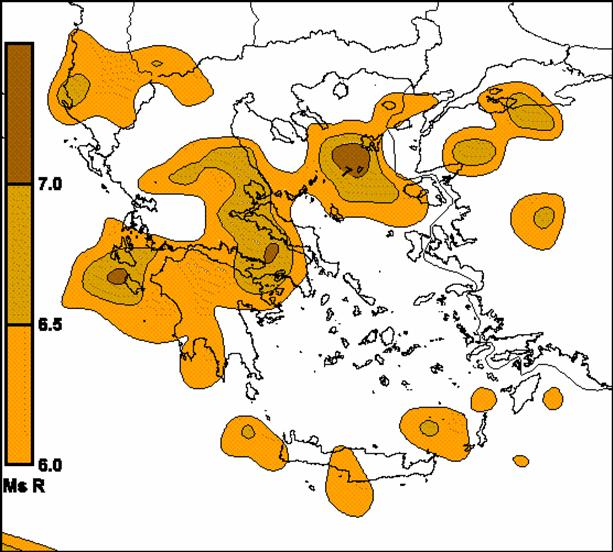Fig. 4.3.7.2.9. The same as above but a threshold of 6R was used.

Year 1985Fig. 4.3.7.2.10. Calculated map, based on data from 1950 to 1985 and expected, potential earthquake magnitudes up to year 1990.

Year 1985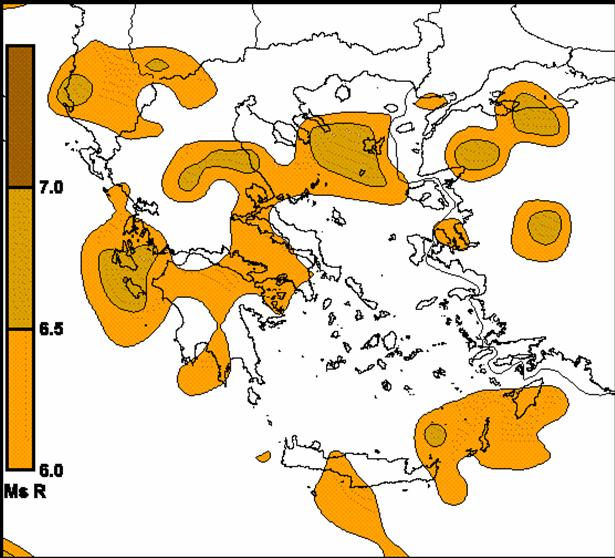Fig. 4.3.7.2.11. The same as above but a threshold of 6R was used.

Year 1990Fig. 4.3.7.2.12. Calculated map, based on data from 1950 to 1990 and expected, potential earthquake magnitudes up to year 1995.

Year 1990Fig. 4.3.7.2.13. The same as above but a threshold of 6R was used.

Year 1995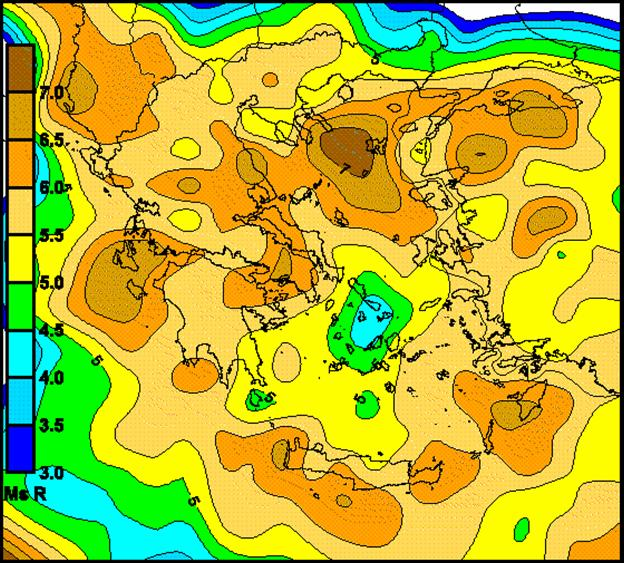Fig. 4.3.7.2.14. Calculated map, based on data from 1950 to 1995 and expected, potential earthquake magnitudes up to year 2000.

Year 1995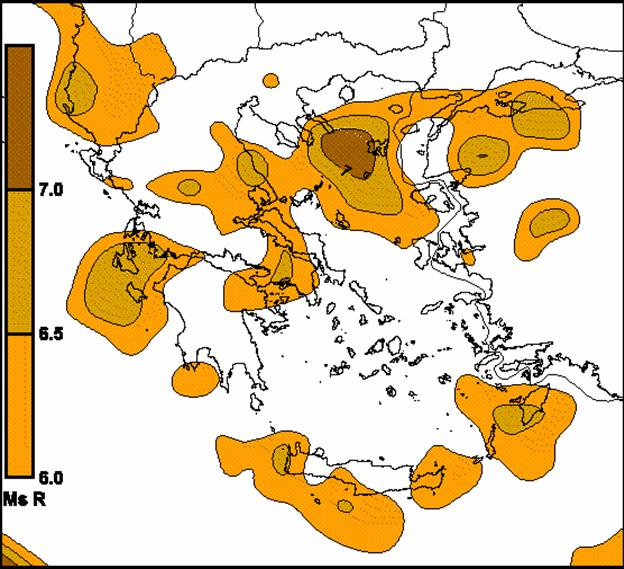Fig. 4.3.7.2.15. The same as above but a threshold of 6R was used.

Year 2000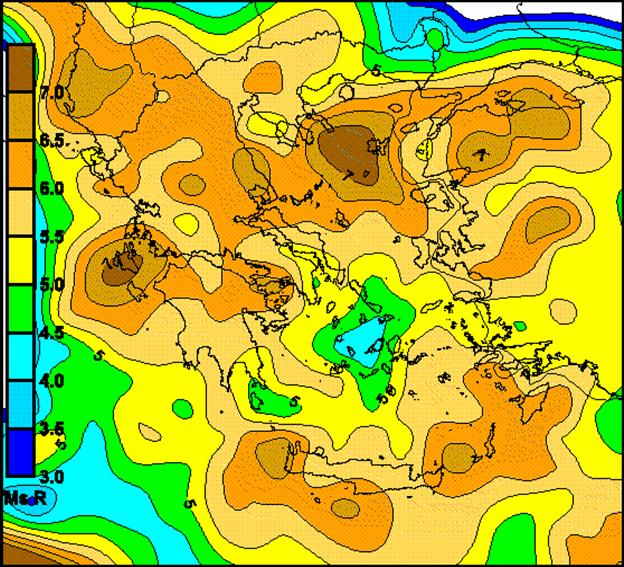Fig. 4.3.7.2.16. Calculated map, based on data from 1950 to 2000 and expected, potential earthquake magnitudes up to year 2005.

Year 2000Fig. 4.3.7.2.17. The same as above but a threshold of 6R was used.

The following maps are presented in order to validate the used method and the results which are obtained through it. On each map, which represents a specific period of time, the corresponding strong earthquakes (Ms >= 6R) which occurred during the following five years were superimposed. The percentage of the earthquakes that occurred, in the predefined area (Ms>=6R), is calculated as a measure of the success of the method. That is the ratio of:

P = EQin / EQtot                                                             (4.3.7.2.3)

Where P is the success percentage, EQin is the number of earthquakes that occurred in the predefined area and EQtot is the total number of the strong EQs that occurred in the specific period of time in the Greek territory.

Period 1970 – 1975Fig. 4.3.7.2.18. Strong seismic events (red circles, Ms> = 6R) during the period 1970 – 1975.

EQtot = 4, EQin = 4, P = EQin / EQtot = 100%

Period 1975 – 1980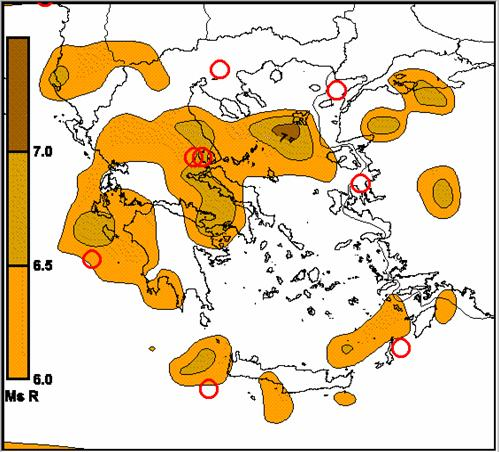Fig. 4.3.7.2.19. Strong seismic events (red circles, Ms>=6R) during the period 1975 –1980.

EQtot = 8, EQin = 5, P = EQin / EQtot = 62.5%

Period 1980 – 1985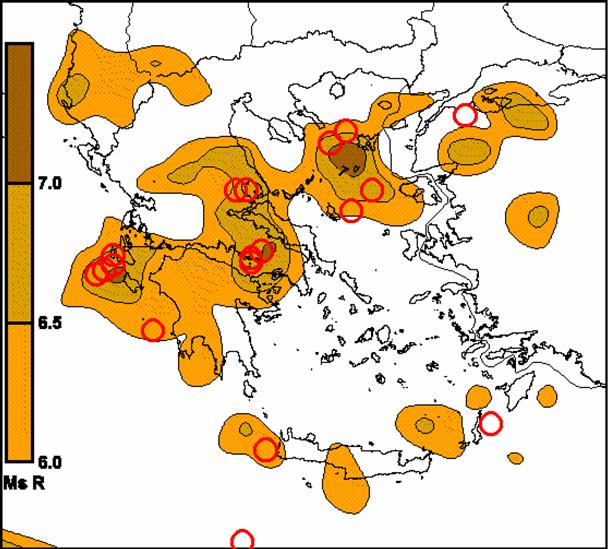Fig. 4.3.7.2.20. Strong seismic events (red circles, Ms>=6R) during the period 1980 – 1985.

EQtot = 17, EQin = 15, P = EQin / EQtot = 88.2%

Period 1985 – 1990Fig. 4.3.7.2.21. Strong seismic events (red circles, Ms>=6R) during the period 1985 – 1990.

EQtot = 3, EQin = 3, P = EQin / EQtot = 100%

Period 1990 - 1995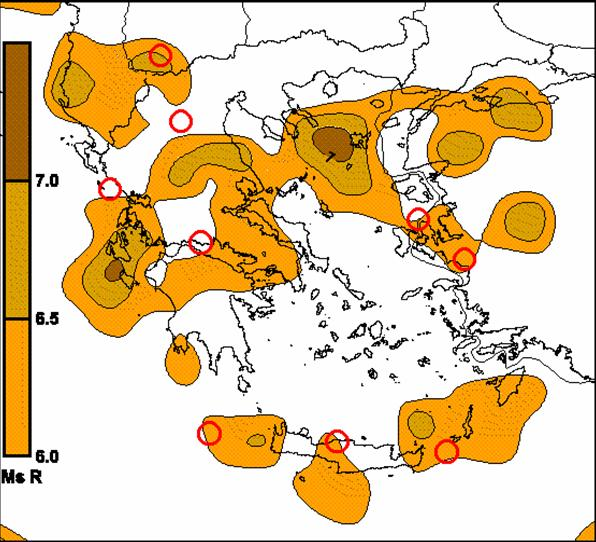Fig. 4.3.7.2.22. Strong seismic events (red circles, Ms>=6R) during the period 1990 – 1995.

EQtot = 9, EQin = 7, P = EQin / EQtot = 77.7%

Period 1995 - 2000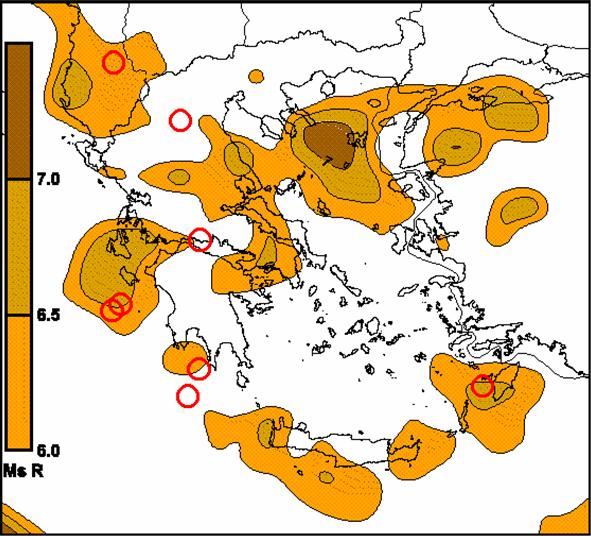Fig. 4.3.7.2.23. Strong seismic events (red circles, Ms>=6R) during the period 1995 – 2000.

EQtot = 8, EQin = 6, P = EQin / EQtot = 75%

Period 2000 - 2003Fig. 4.3.7.2.24. Strong seismic events (red circles, Ms>=6R) during the period 2000 – 2003.

For the most recent period (2000 – 2003), the (P) value has not been calculated, since the seismic activity is still in progress and two more years have to elapse.C. Discussion – Conclusions.

The Greek territory, considered as a unit area, appears to be charged with seismic energy, continuously, since 1950. This is the result of the application of the energy flow model that indicates a continuous decrease, in total, of the seismic energy release in the Greek area. The latter, is demonstrated, in figure (4.3.7.2.2), where the energy flow rate (EFL) values decrease, along time, since 1950 and consequently, excess seismic energy is stored in the lithosphere. Therefore, it is expected that, some time in the future, the stored, seismic energy will be released through the occurrence of some strong seismic events.
The detailed study of the prepared maps (fig. 4.3.7.2.4 – fig. 4.3.7.2.17), for consecutive periods of 5 years (1970 – 2000), reveals the dynamic character of the phenomenon. A significant increase of seismic, potential charge is observed even in a five years period.
The observed, increased values of the seismic potential, in all the prepared maps, are distributed along the axis of the North Anatolian Fault Zone and the Southern Aegean Seismic Arc. The latter, complies with the seismological observations which concern the spatial distribution of the strong seismic events in the Greek territory.
The periods of time, when the seismic potential has reached large levels, is indicated by expected earthquake magnitudes of levels above seven (7R). A very good example of the latter is demonstrated in figure (4.3.7.2.20) for the period 1980 – 1985. The prepared map for the year 1980 indicates the presence of three centers of large seismic potential (Ms >7R, Kefalonia island, Korinthos – Alkyonides – Thiva area, Limnos island). In the next five years (1980 – 1985) to follow, 17 strong (M>6R) seismic events occurred, releasing a significant amount of seismic energy. The latter is made clear by the next prepared map (fig. 4.3.7.2.21, 1985) and the small number of strong seismic events that occurred (only 3) in the following five years period (1985 – 1990).
What is more important, as a result of comparing the maps to each other, is the fact that a period of large seismic activity discharges the Greek territory for only .5 – 1.0 R. Therefore, distinct areas can be considered as being at a state of continuous, large seismic charge (expected EQ levels up to 6R) and slight, occasional increases of stress, trigger strong seismic events in the same areas.
In all these prognostic maps, which were prepared for the years 1970, 1975, 1980, 1985, 1990, 1995, 2000, were superimposed the corresponding large (Ms>6R) EQs for the next five years period of time. The percentage of success ranges from 62.5%, for the period 1975 – 1980, to a value of 88.2%, which was achieved for the period 1980 – 1985, in a total of 17 strong EQS, which occurred during this period. Obtained values of 100% for the percentage of success are based in a small number of strong seismic events and could be no reliable at all. Nevertheless, these seismic events still verify the validity of the method, used for the calculation of these seismic, potential maps.
As far as it concerns the small number of the seismic, strong events that didn’t occurred in the predefined areas, it could be attributed to errors in compilation of these maps. A major error can be introduced by inadequate, available seismic data for the calculation of the cumulative energy graph (Ec) vs. time, for each frame, used, during the application of the lithospheric energy flow model.
The calculated seismic potential maps, for different periods of time, were compared with the Athens EQ (1999, Ms = 5.9R), Karpathos (2001, Ms = 6.6R) and Zakynthos-Kefalonia EQ (2003, Ms = 5.8R) strong seismic events in Greece.
In the following figure (4.3.7.2.25), the seismic potential map of Greece, for the year 1995, is shown, along with the strong (Ms >6R) seismic events that occurred in the period 1995 - 2000. The red arrow indicates the place where the Athens EQ occurred (Ms = 5.9R). It coincides with the narrow area, of expected events of Ms >6.5R, observed, in the same place. A comparison of this map with the one in figure (4.3.7.2.22), compiled for the year 1990, indicates that seismic potential at the regional area of Athens was built-up during the time span of 1990 – 1995.Fig. 4.3.7.2.25. Seismic potential spatial distribution in Greece is presented for the year 1995. The red arrow indicates the location of Athens (1999) EQ.

A comparison of figure (4.3.7.2.25) with figure (4.3.7.2.24), of the seismic potential for the year 2000, reveals that there is still accumulated, large seismic potential, at a rather short distance, west of Athens.
The next example concerns Karpathos strong EQ (2001, Ms = 6.6R). The corresponding map of the seismic potential was compiled for the year 2000. The red arrow indicates the location of the earthquake. The coincidence of its epicenter with the area of increased, seismic potential (expected EQ with Ms >6.5R) is more than evident.Fig. 4.3.7.2.26. Seismic, potential spatial distribution, in Greece is presented, for the year 2000. The red arrow indicates the location of Karpathos (2001) EQ.

The last example refers to Zakynthos - Kefalonia (2003, Ms = 5.8R). The red arrow indicates the location of this EQ. Even if this EQ is not of a magnitude larger than 6R, it is still considered as a rather strong one and of significant magnitude since it occurred near inhabited areas.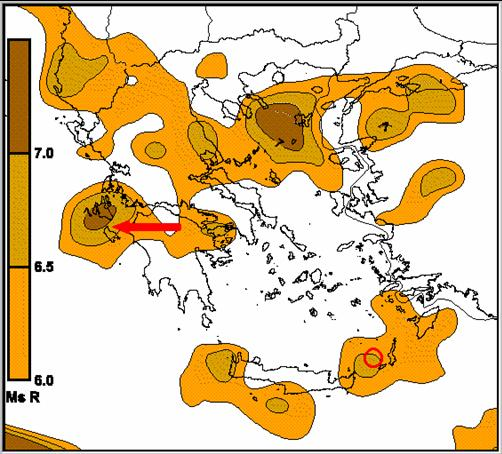Fig. 4.3.7.2.27. Seismic, potential spatial distribution in Greece is shown for the year 2000. The red arrow indicates the location of Zakynthos - Kefalonia (2003) EQ.

Apart from the previous, presented examples, a statistical study was applied on the, obtained, results, as follows:

a. the total number of strong seismic events, during the period 1970 – 2000, is equal to 50.

b. the total number of the seismic events that occurred, in the predefined as seismically active areas, is 41.

c. For the total period between 1970 – 2000 the P value is calculated as:

P = 41/50 = 82%

For the last compiled map (fig. 4.3.7.2.24), for the year 2000, it is not possible to calculate a P value, since two more years are left for completing the five years time interval, needed. Therefore, only a statistical extrapolation, as follows, can be made, based, on the previous results.
Since only one strong earthquake occurred in the period 2000 – 2003, and assuming the value of 82% is the correct one, then 5 more strong EQs are expected, within the next two years, four of them must occur in the predefined areas, so that the P value is: P = 5/6 = 83% that is very close to the calculated 82% (average value, calculated, for the entire time period of the study), and it is the result of the smallest pair of integer numbers found.
If another scenario is followed, for a minimum P value found of 62.5% (period 1975 – 1980), then, by following the above procedure, it is found that four more strong EQs must occur, two of them in the predefined area, so that the P value is: P = 3/5 = 60% and it is very close to the assumed value of 62.5%. Regardless the used statistical hypothesis, 4 – 5 strong (Ms > 6R) seismic events are, theoretically, expected, to occur within the next period of time up to 2005, included (Thanassoulas et al. 2003).
Four years have elapsed, at the time of writing this book, since the time (2003) the latter scenarios had been presented. Therefore, it is possible to present the entire 2000 – 2005 seismic potential map and to compare it with the “guesses”, made in 2003. This is shown in the following figure (4.3.7.2.28).Fig. 4.3.7.2.28. Strong seismic events (blue circles, Ms>=6R) during the period of time 2000 – 2005.

The number of strong (M > 6R) seismic events that took place is seven (7) and four (4) of them are located in seismically charged areas. The calculated P value is:

P = 4/7 or 57%

If the double, seismic event of Hios Island (eastern Greece), is considered as of marginal success, then the P value becomes:

P = 6/7 or 86%

Actually, both hypothetical scenarios were superceded by a larger number of earthquakes (6) verifying thus the number of expected strong EQs in the period 2000 – 2005.
Finally, the compiled map for the year 2000, that is the current map used for the seismic potential evaluation of the period of time 2000 – 2005, is compared with the seismicity in Greece (April, 2003, Ms > 4.5 R). This map is presented in the following figure (4.3.7.2.29).
In this map, the black solid lines indicate the location of the deep fracture zones of the lithosphere. The red circles indicate the location of the earthquakes of magnitude Ms > 4.5R that occurred during the period 1st April – 24th April 2003.
It is clearly shown that, the epicenters coincide with the predefined areas, where intense seismic activity is expected in the future. Moreover, these epicenters are very closely located to the deep fracture zones of the lithosphere, while the predefined areas of peak large seismic potential are closely related to the deep fracture lithospheric zones.Fig. 4.3.7.2.29. Seismic, potential map is shown, for the year 2000, along with the April, 2003 seismicity (red circles) with Ms > 4.5 R.

It is worth to compare the current, seismic hazard map (fig. 4.3.7.2.1), compiled by seismological data only, with the seismic, potential maps, compiled, by the application of the energy flow model of the lithosphere. The latter indicates, in medium term periods of time, the dynamic change of the seismic potential charge of the lithosphere and therefore, it can be used so that medium term measures against any seismic event, can be taken, by the State Authorities, in areas prone to intense, seismic activity.
Consequently, the seismic hazard map of Greece should be recompiled more frequently, since it changes dynamically, in medium time intervals, along the time span.